NCERT Exemplar Solutions for Class 7 Maths Chapter 7 Comparing Quantities are available here. Students can check for the solutions whenever they are facing difficulty while solving the questions from NCERT Exemplar Solutions for Class 7. These solutions are available in PDF format so that students can download it and learn offline as well. This book is one of the top materials when it comes to providing a question bank to practice.

Chapter 7 – Comparing Quantities is defined as a ratio, which is a comparison between two or more like quantities in the same units. This suggests that a ratio can be simplified by dividing its terms by the same number. Therefore, this suggests that the terms of a ratio can be multiplied by the same number. Now, let us have a look at some of the concepts discussed in this Chapter.

• Converting fraction numbers to a percentage
• Converting Decimals to Percentage
• Converting percentage to Fraction or Decimals
• Increase or Decrease as Percent
• Price Related to an Item or Buying and Selling
• Profit or Loss as a Percentage
• Interest for Multiple Years

## Download the PDF of NCERT Exemplar Solutions for Class 7 Maths Chapter 7 Comparing Quantities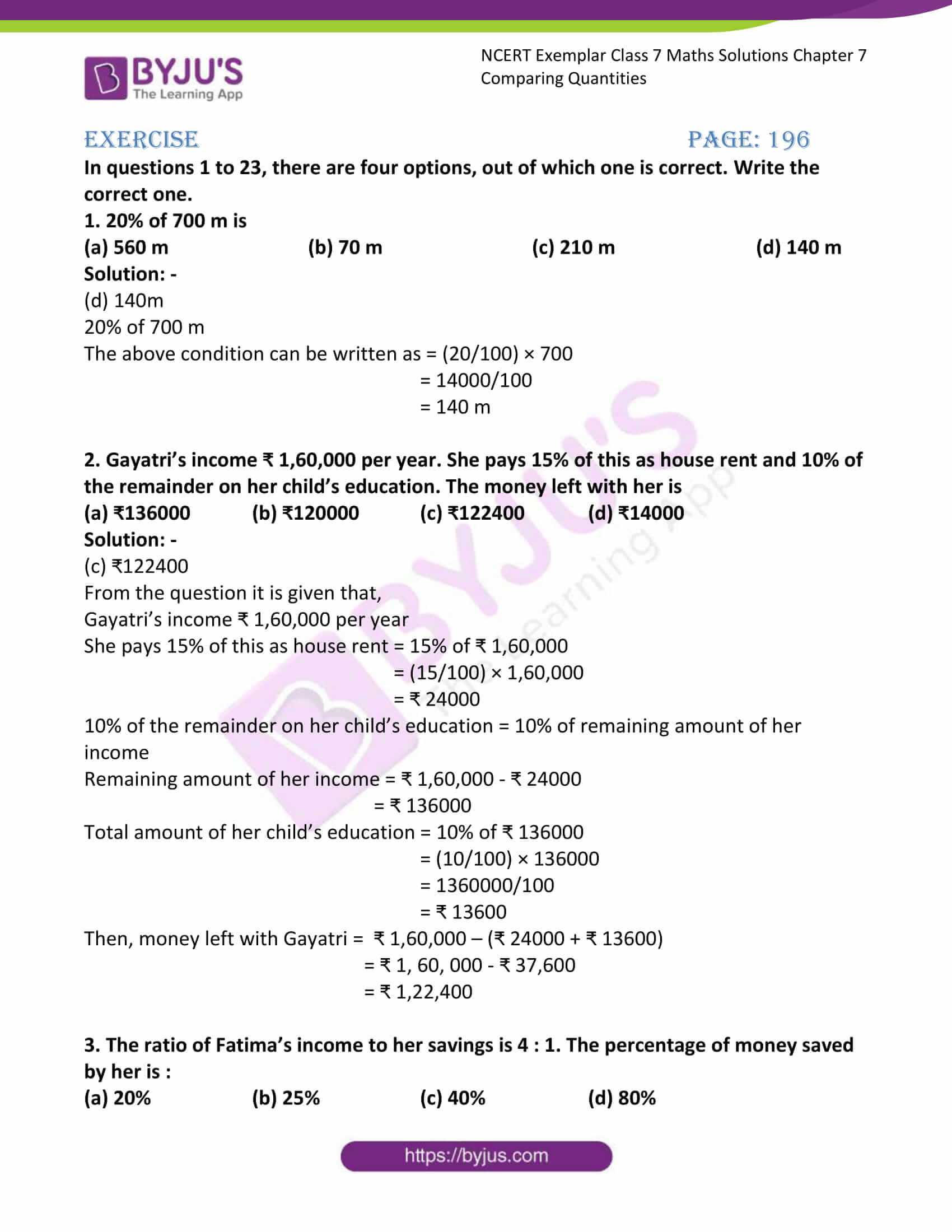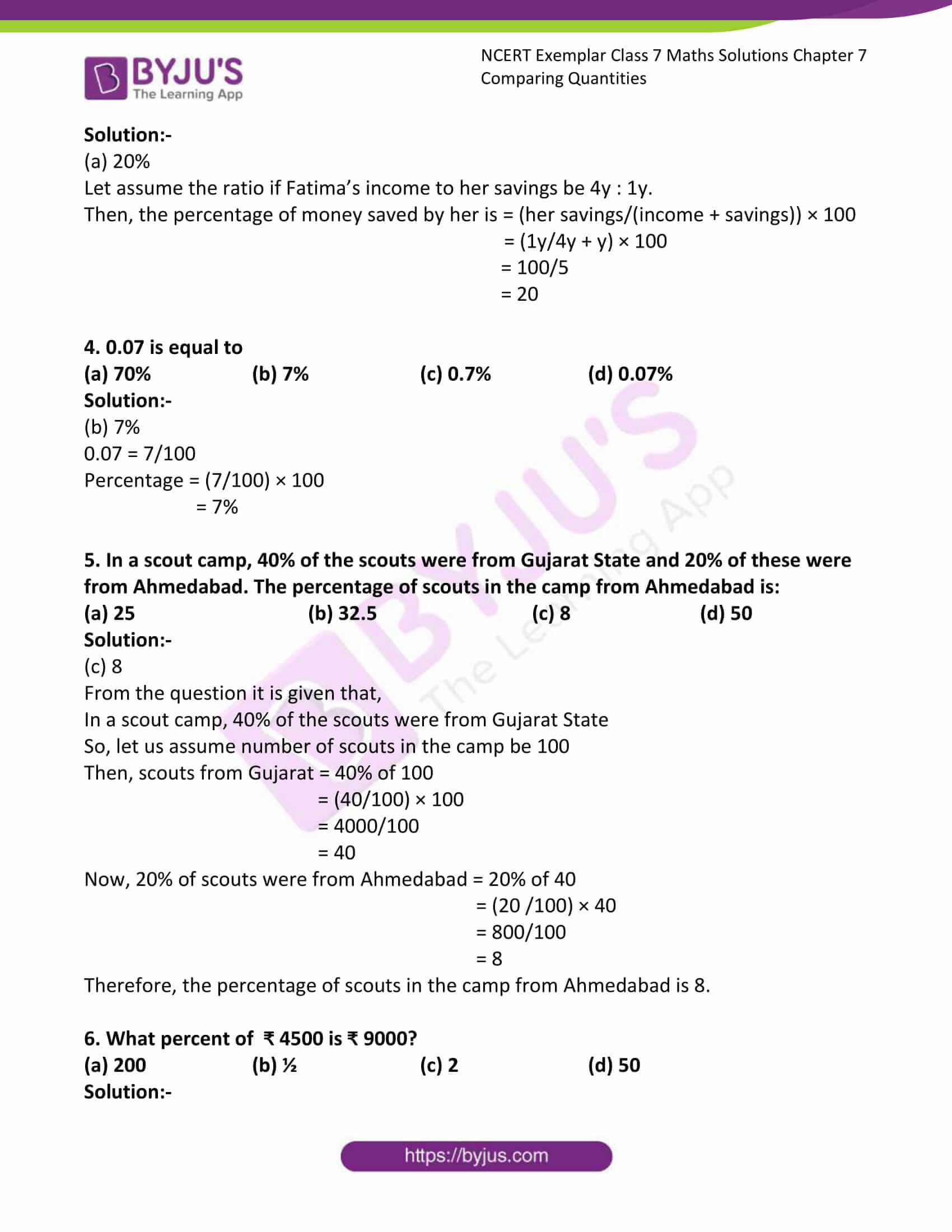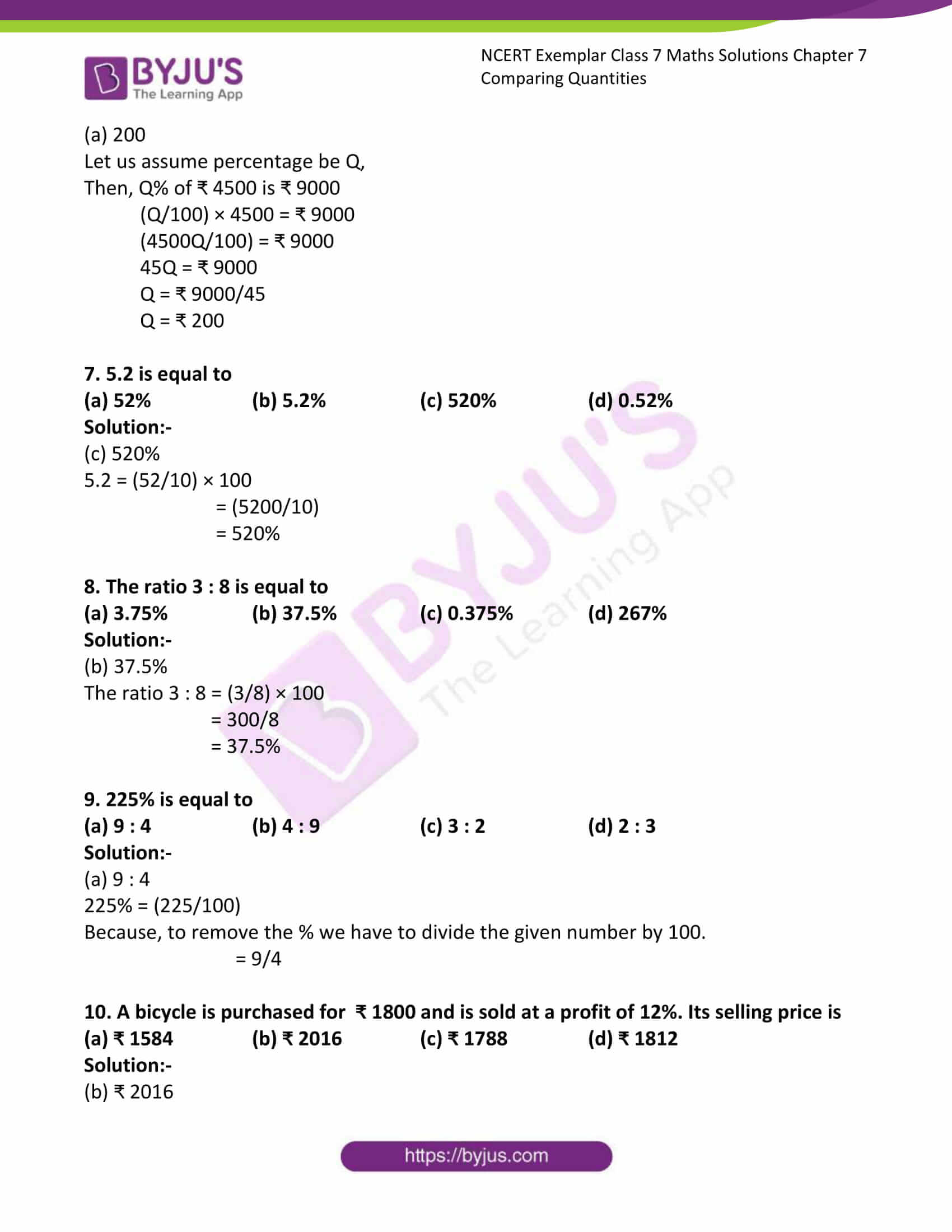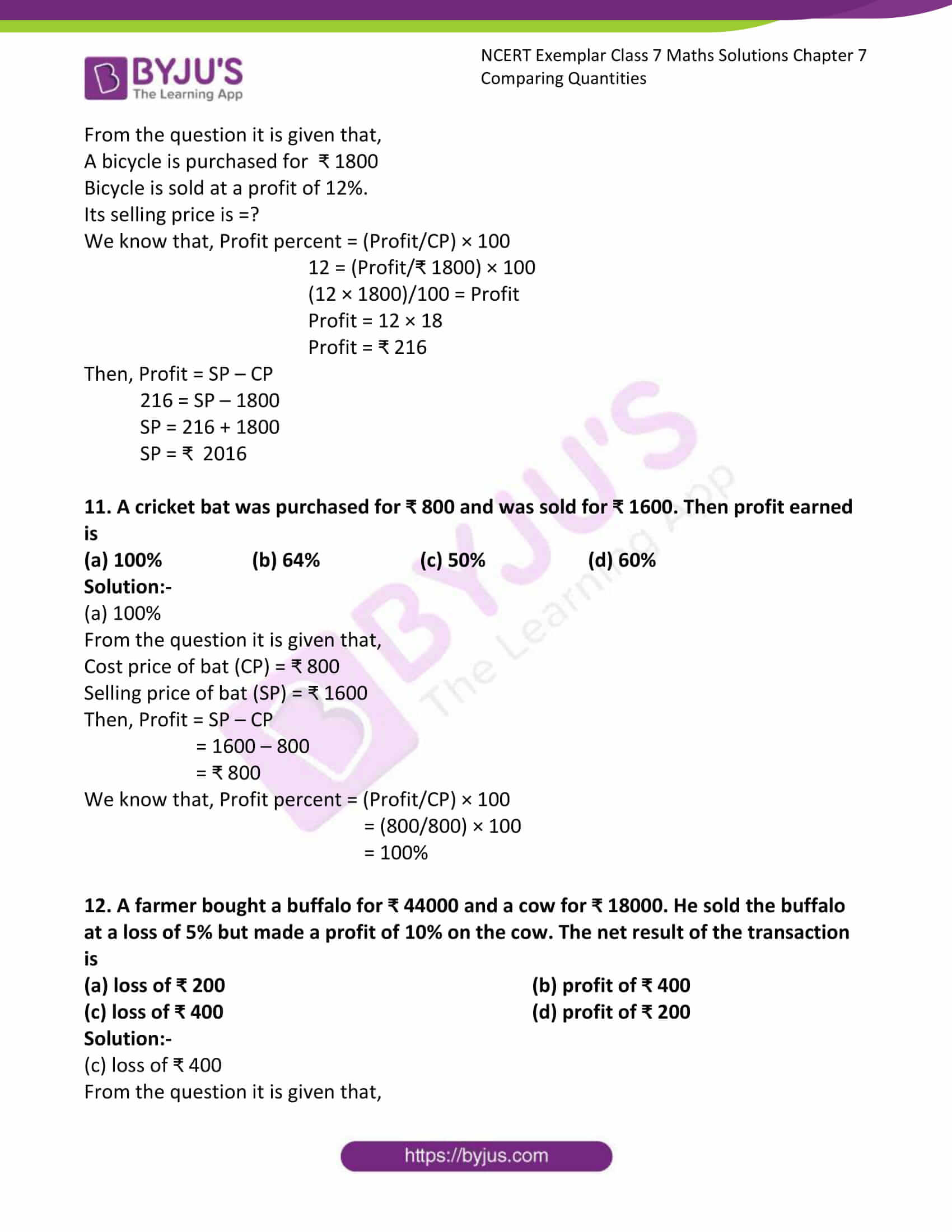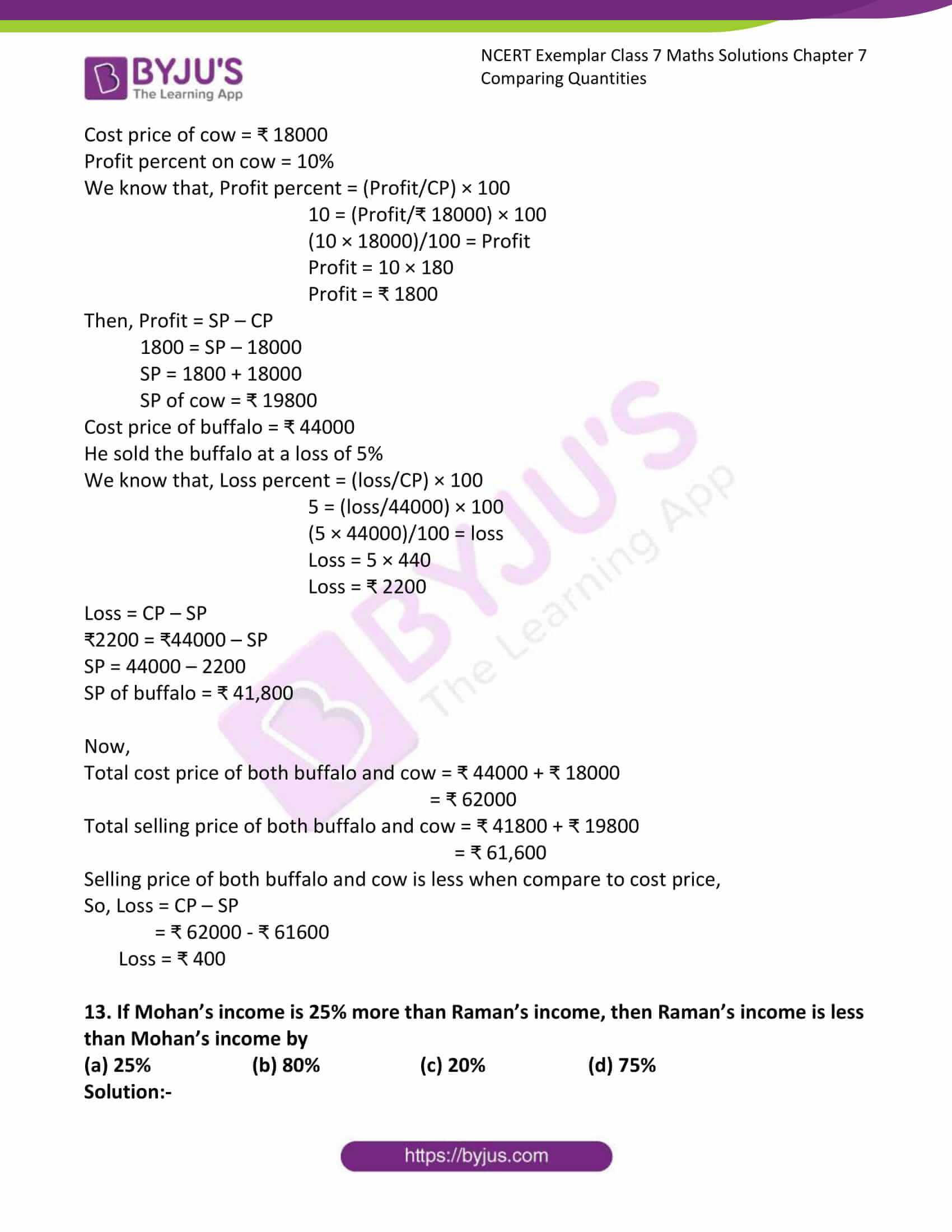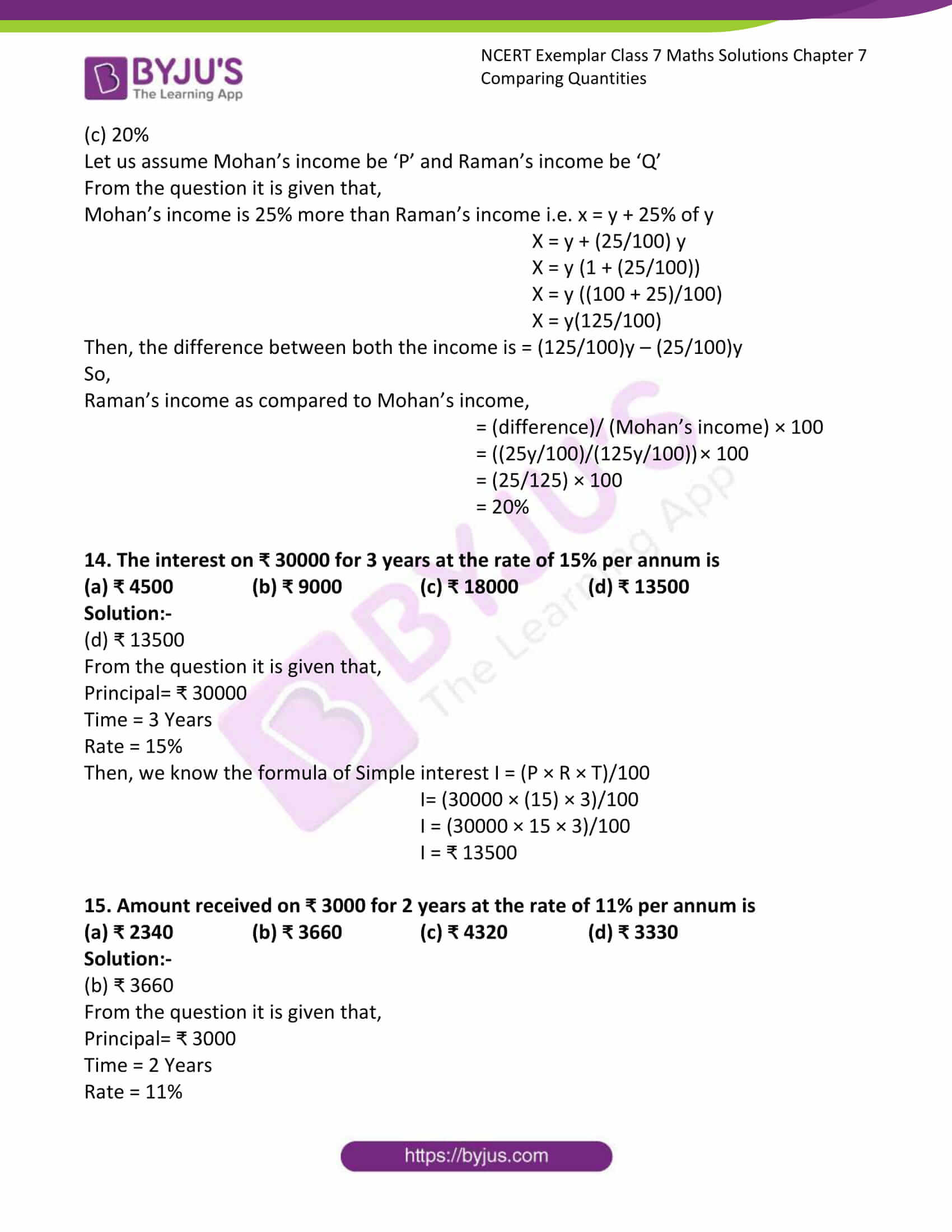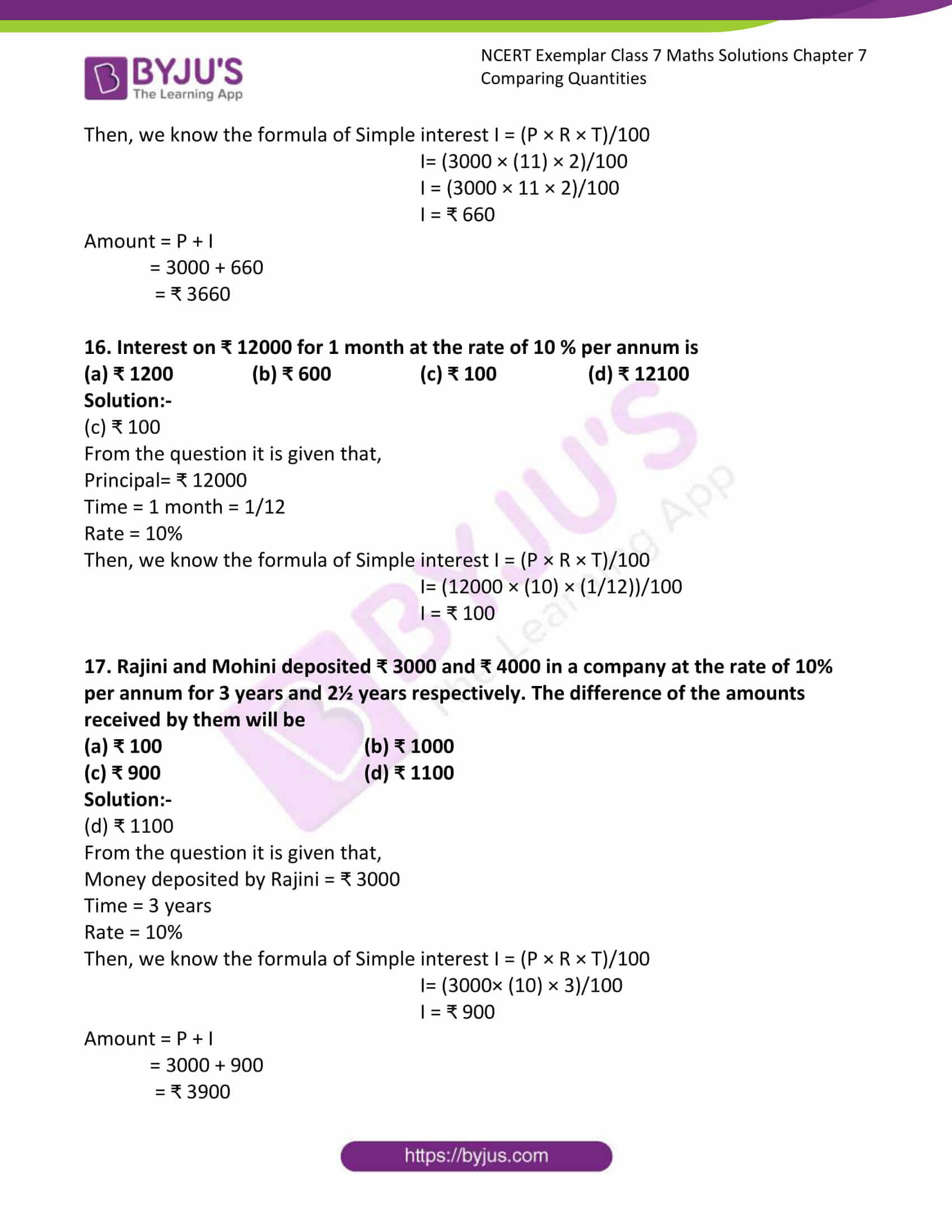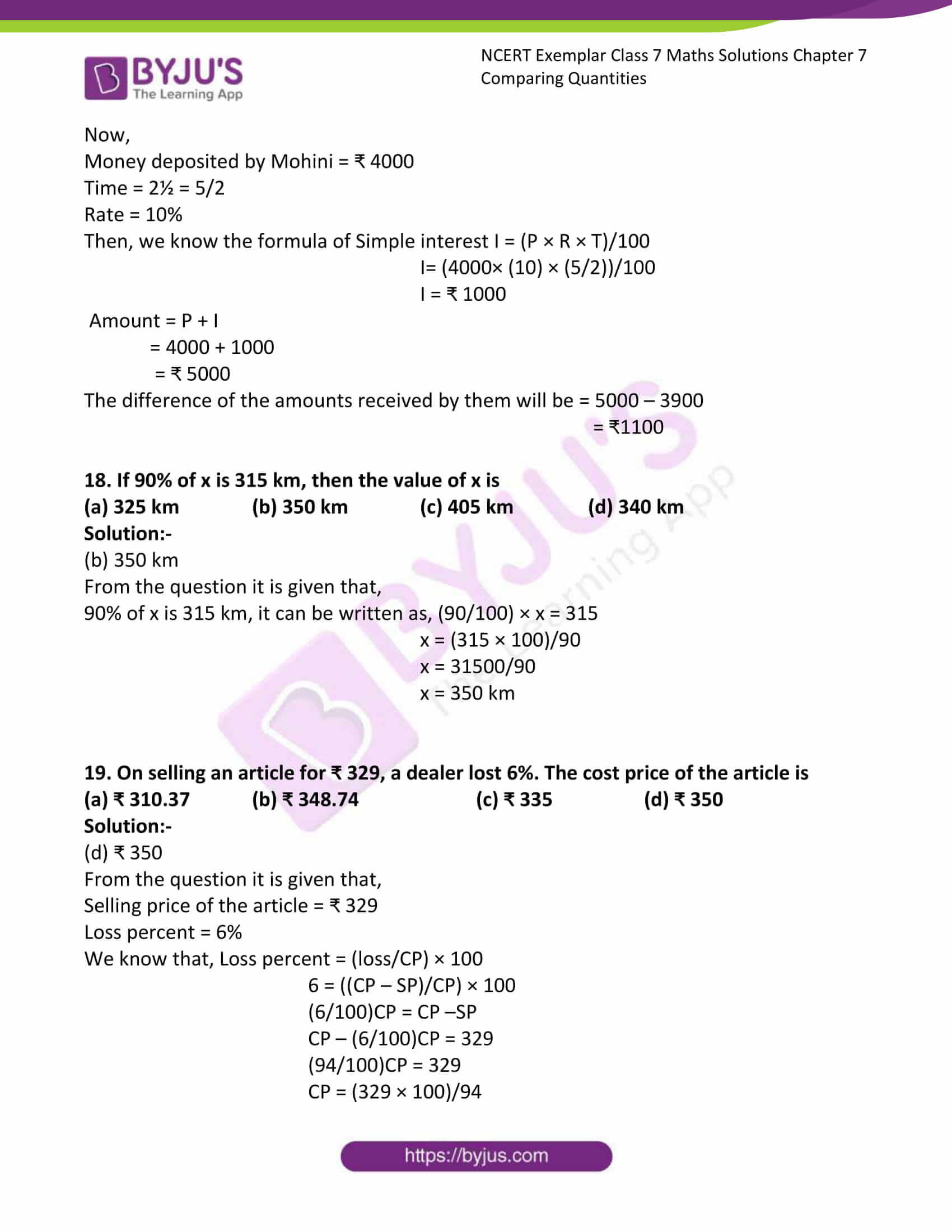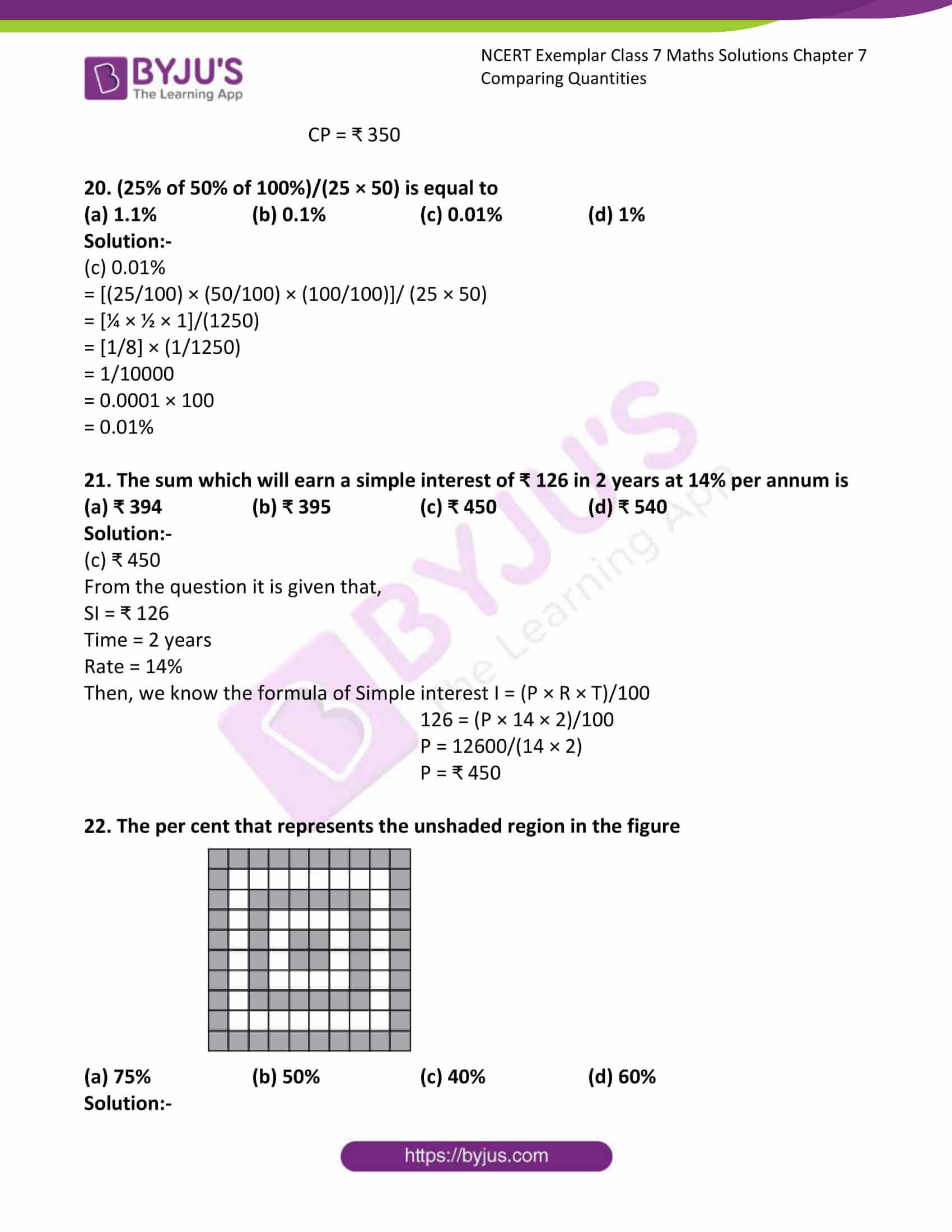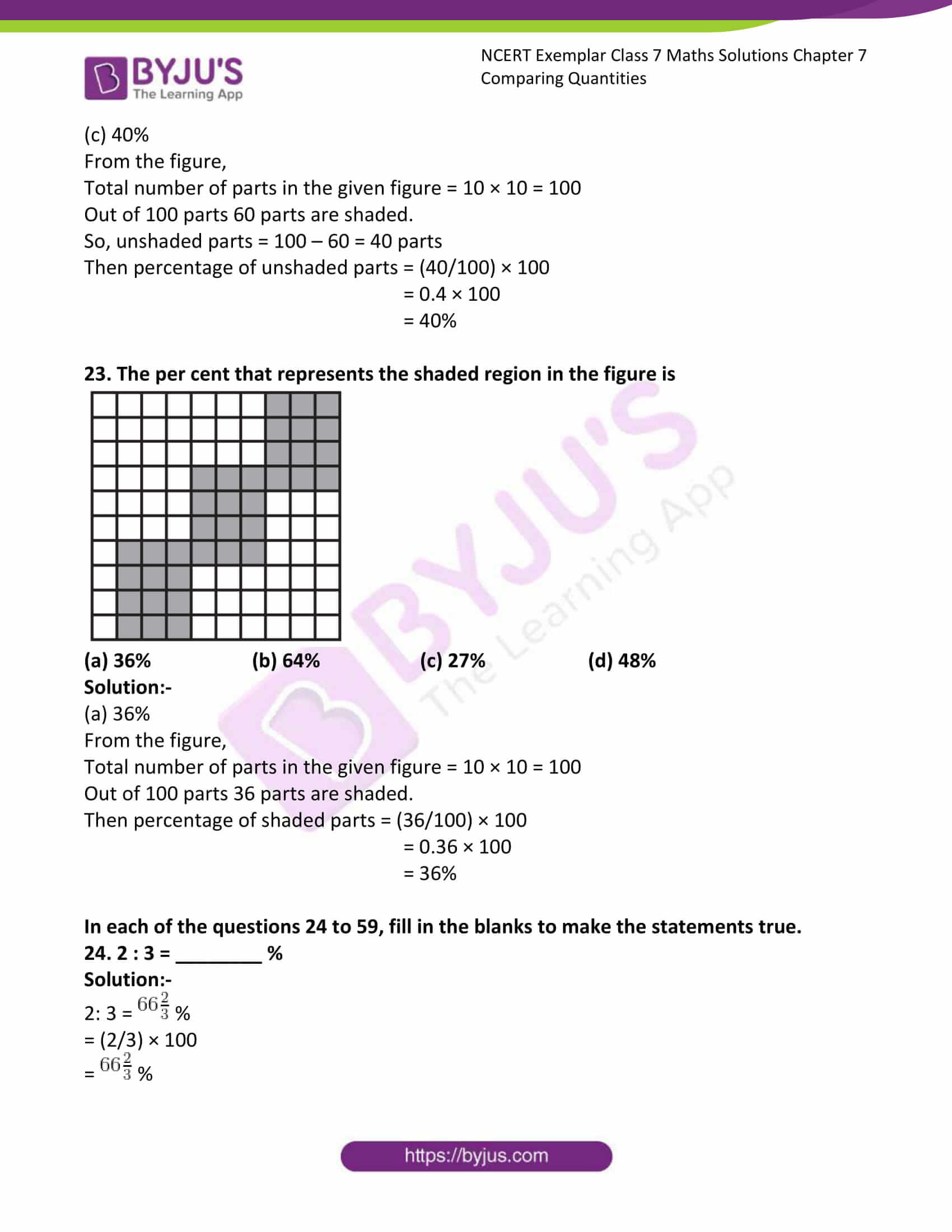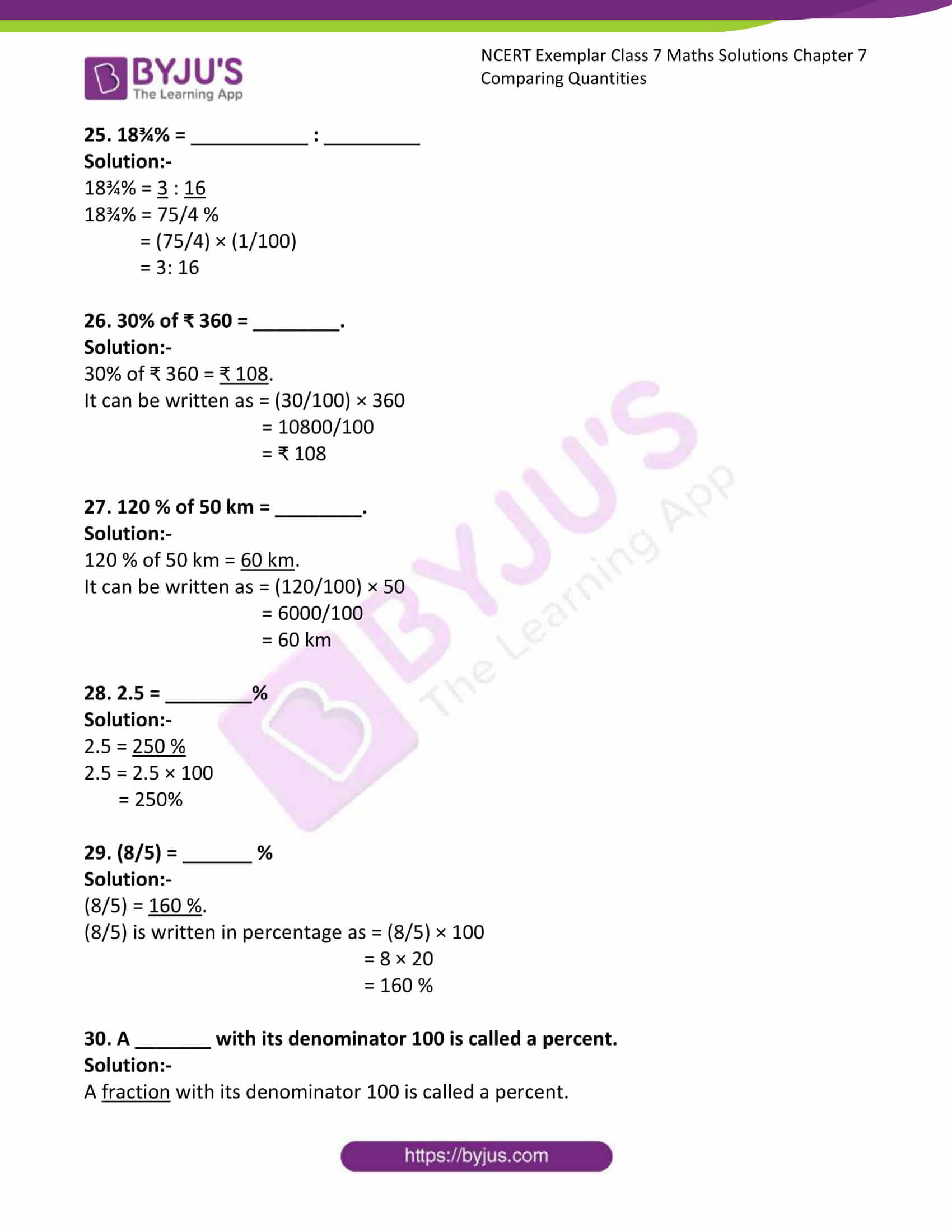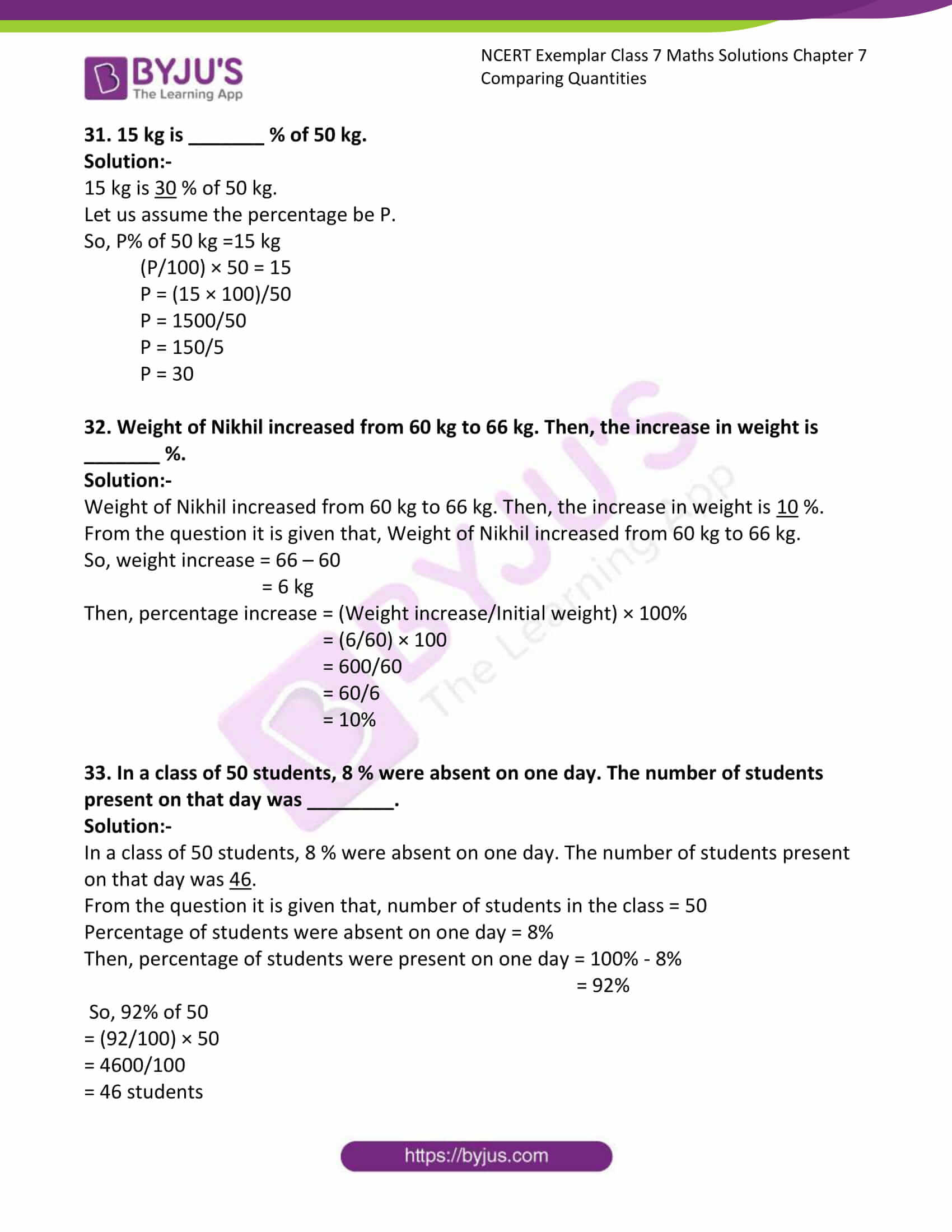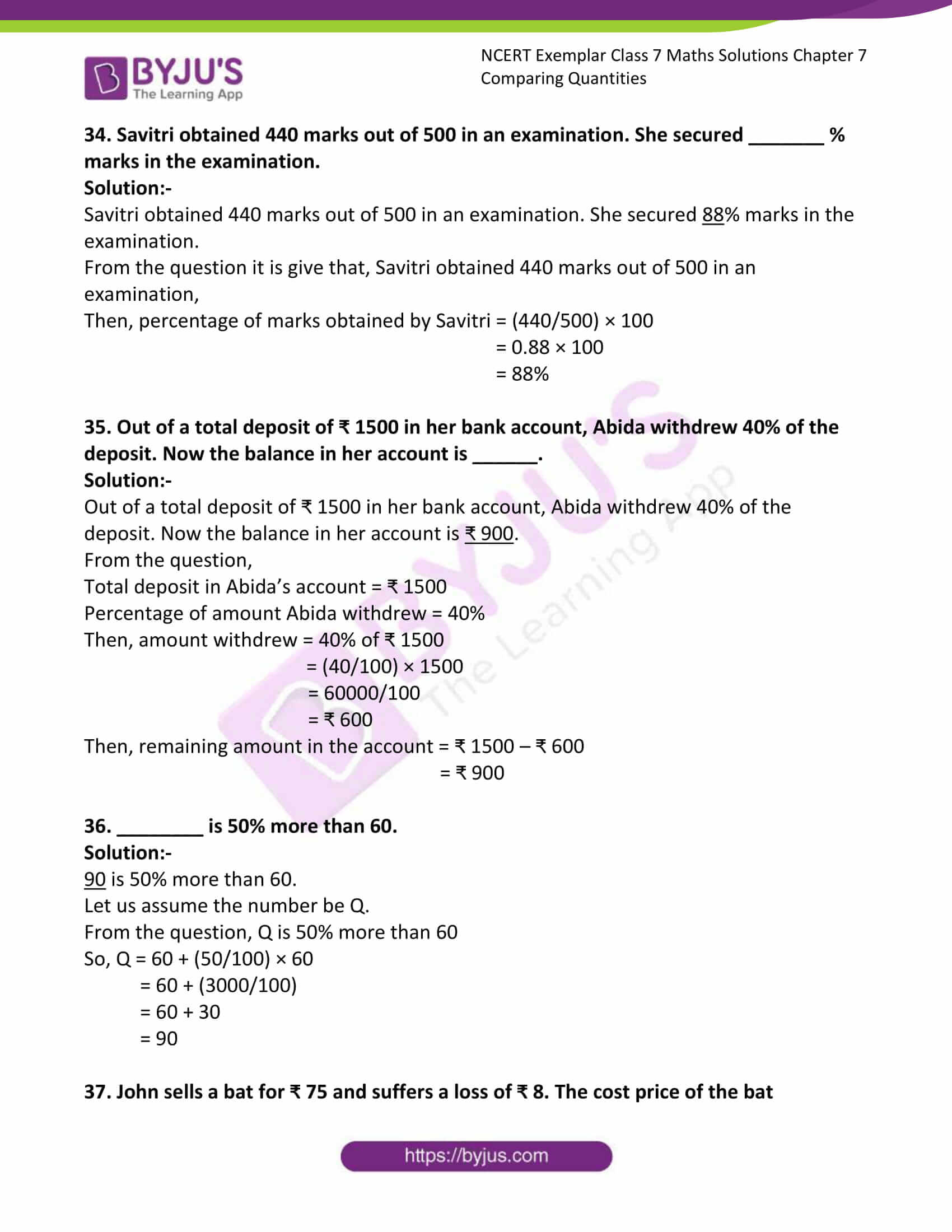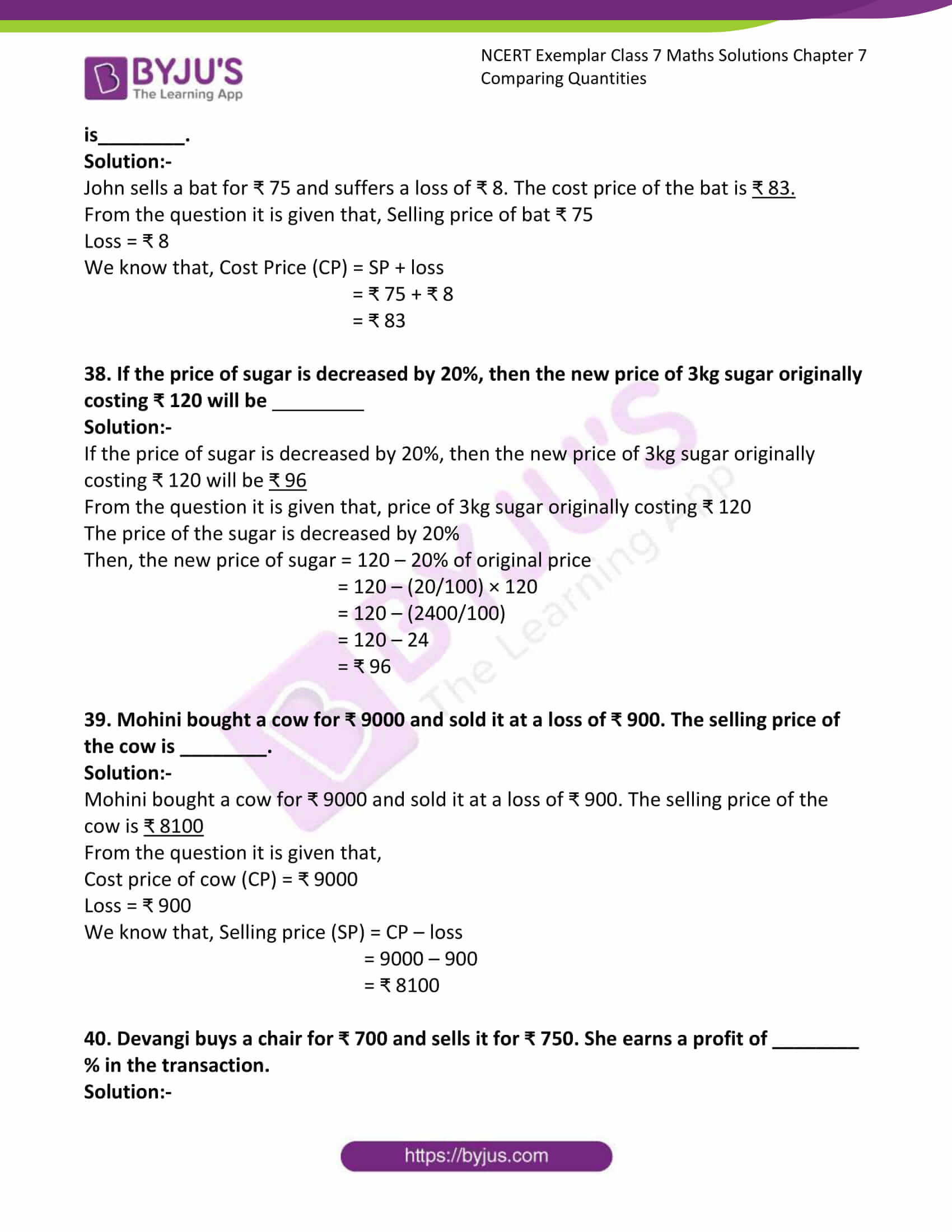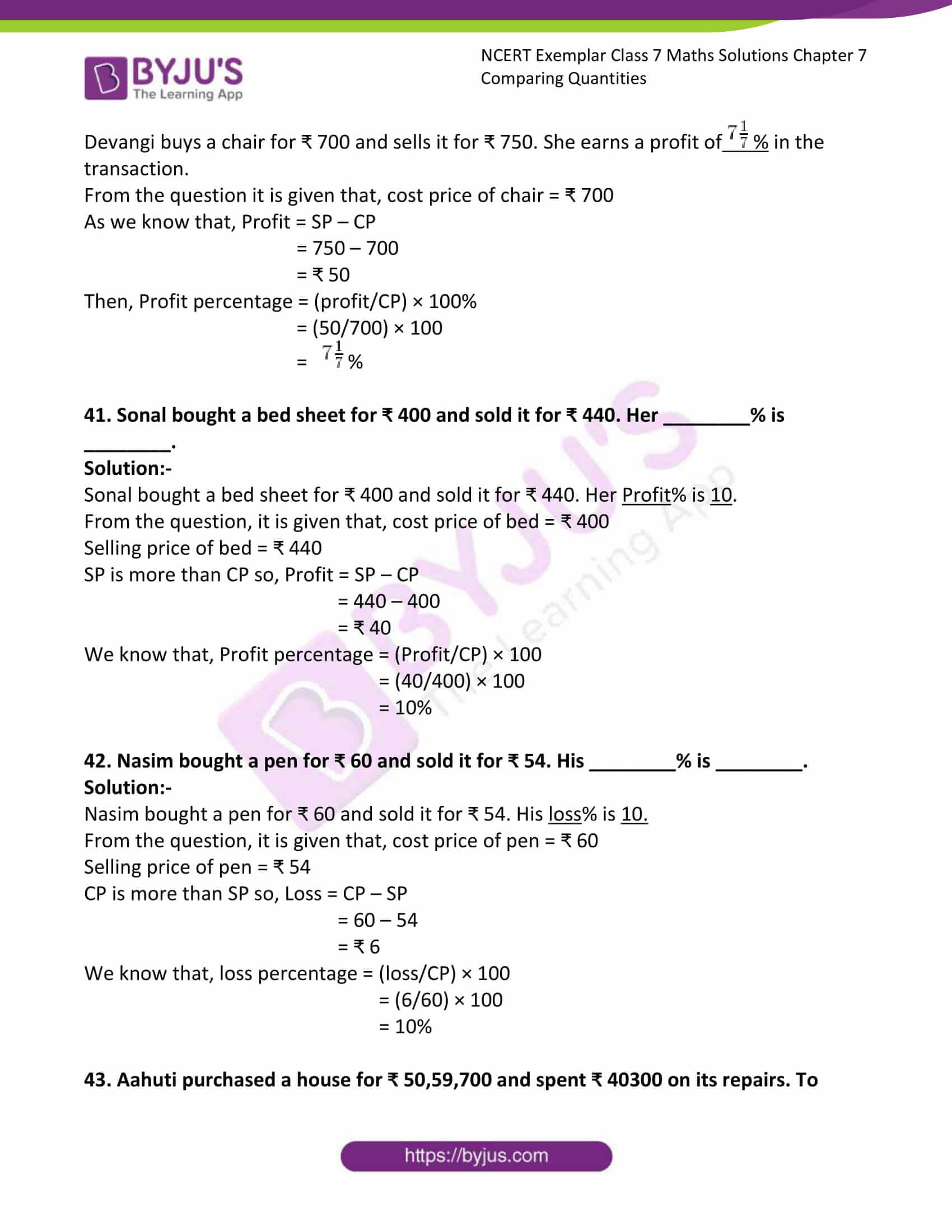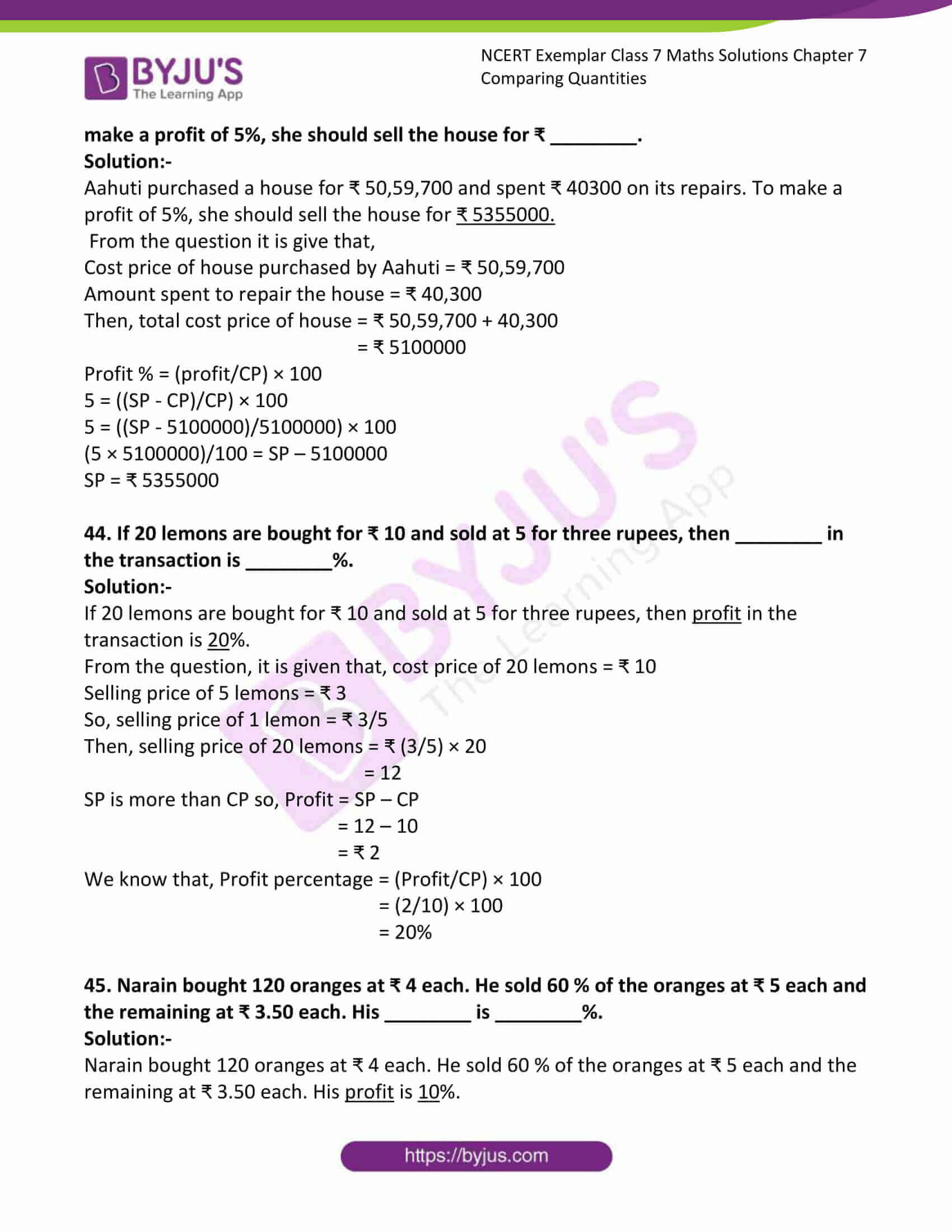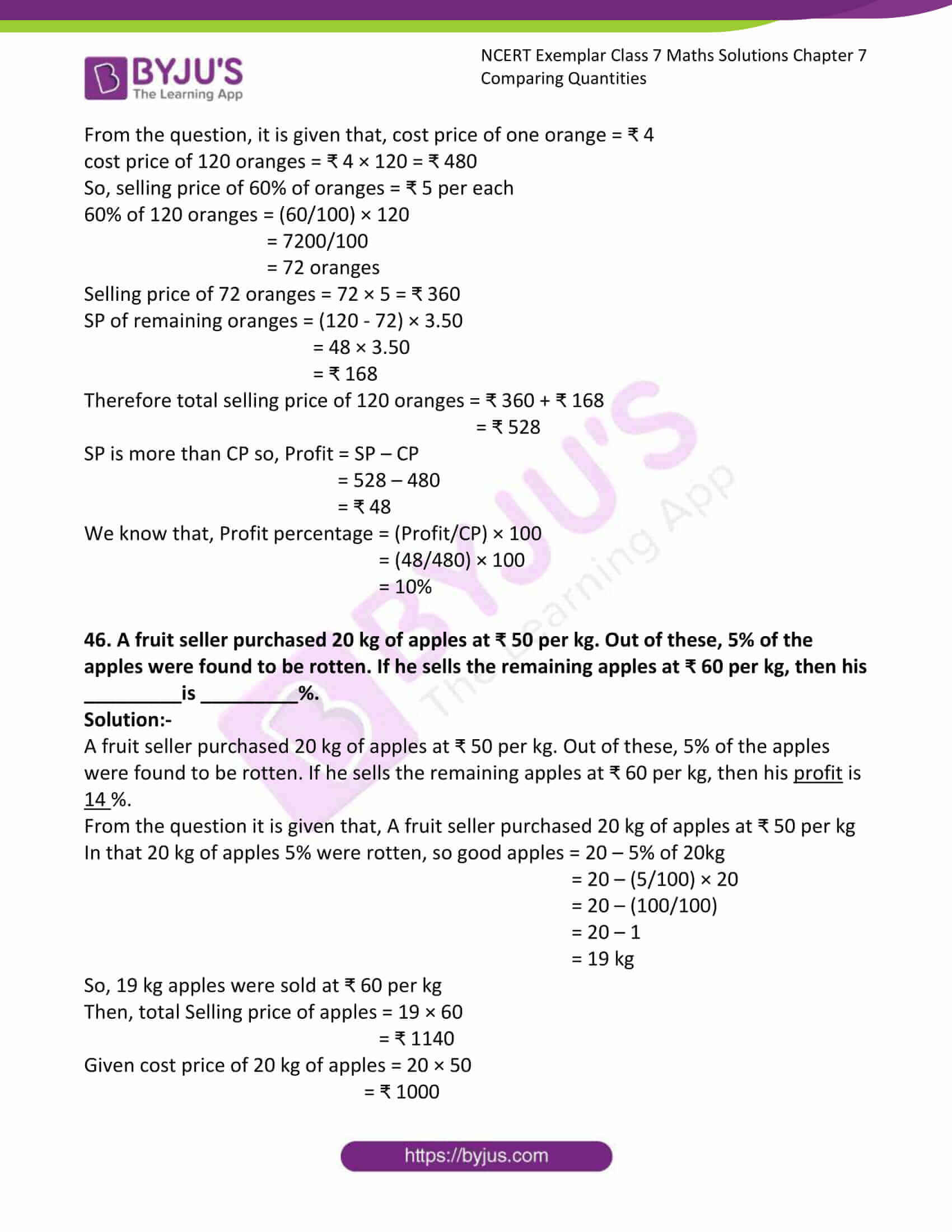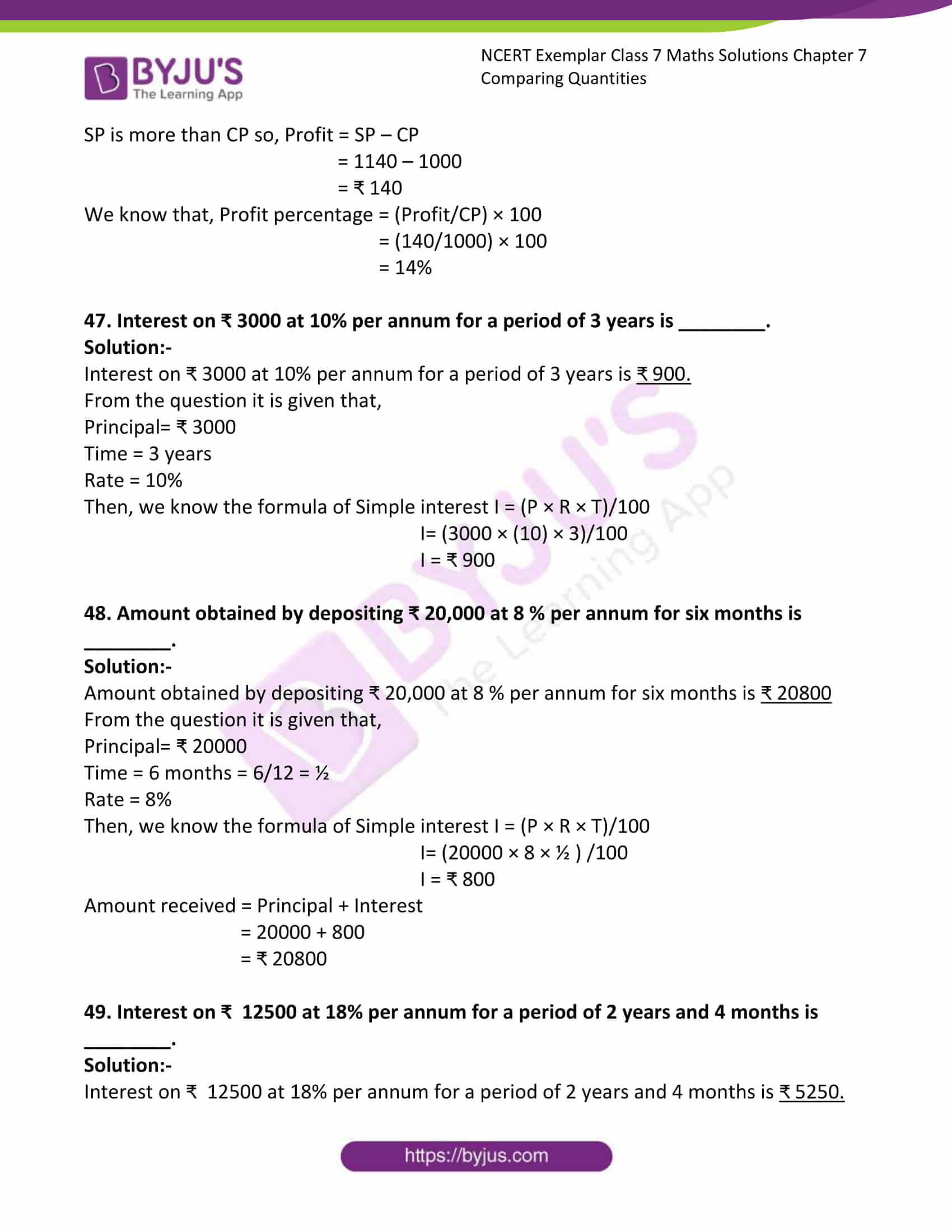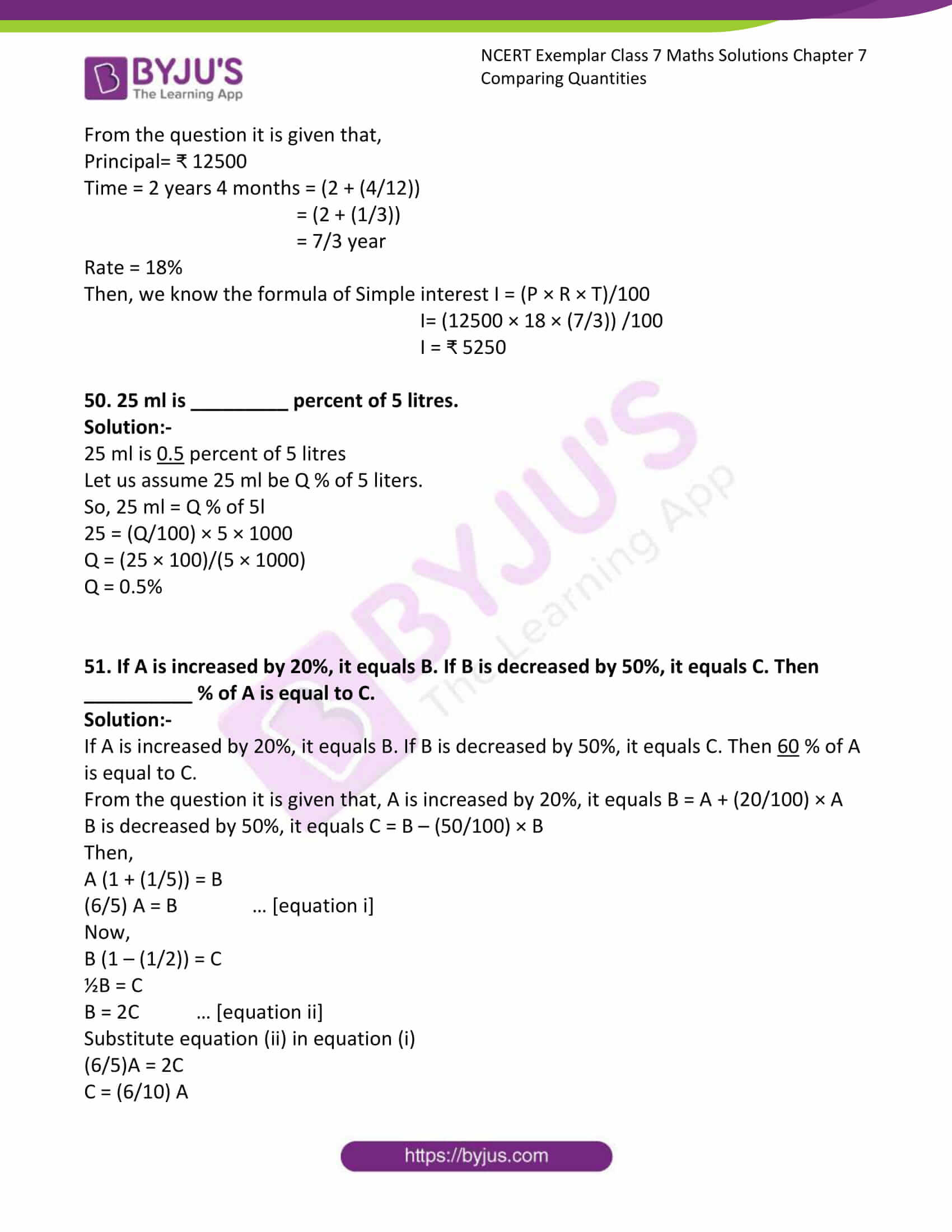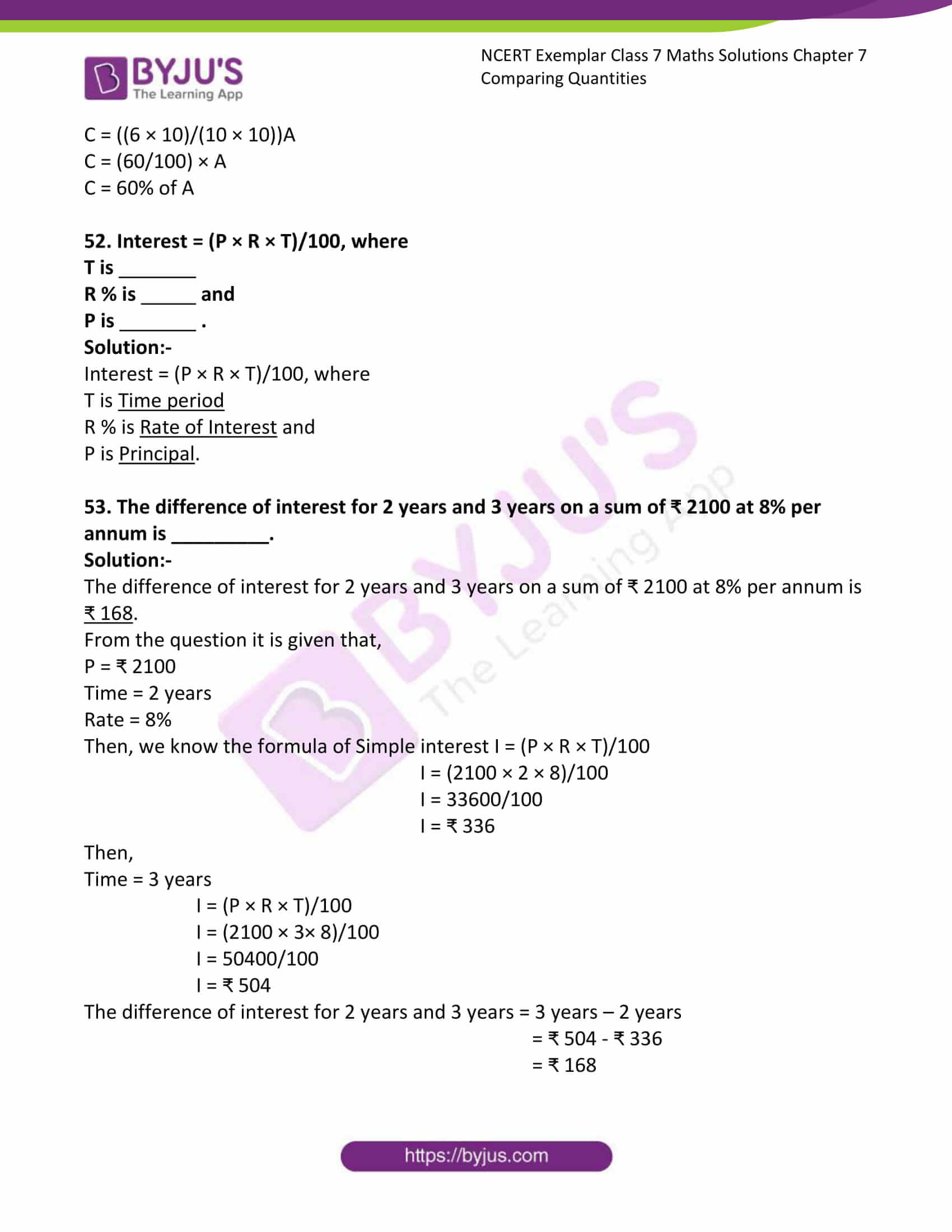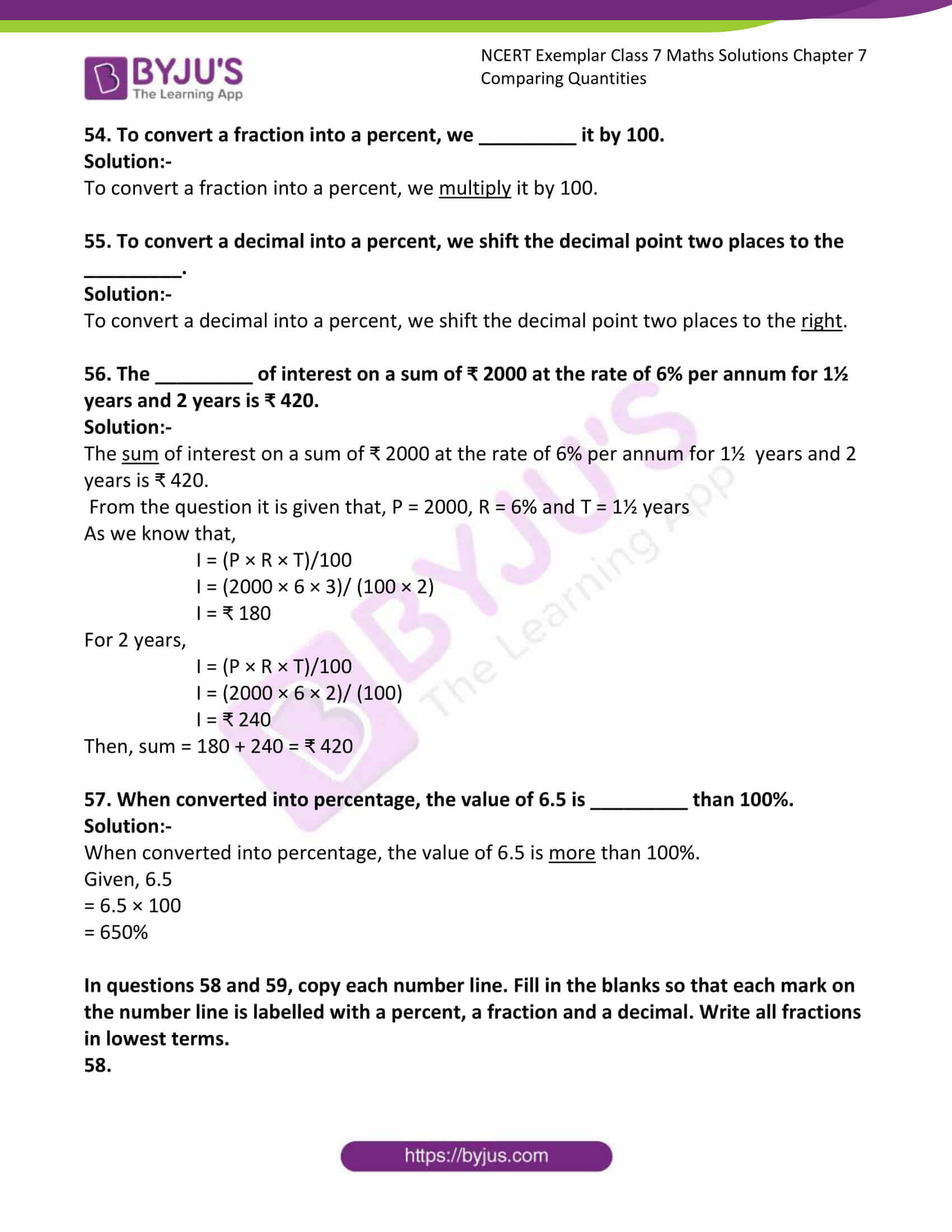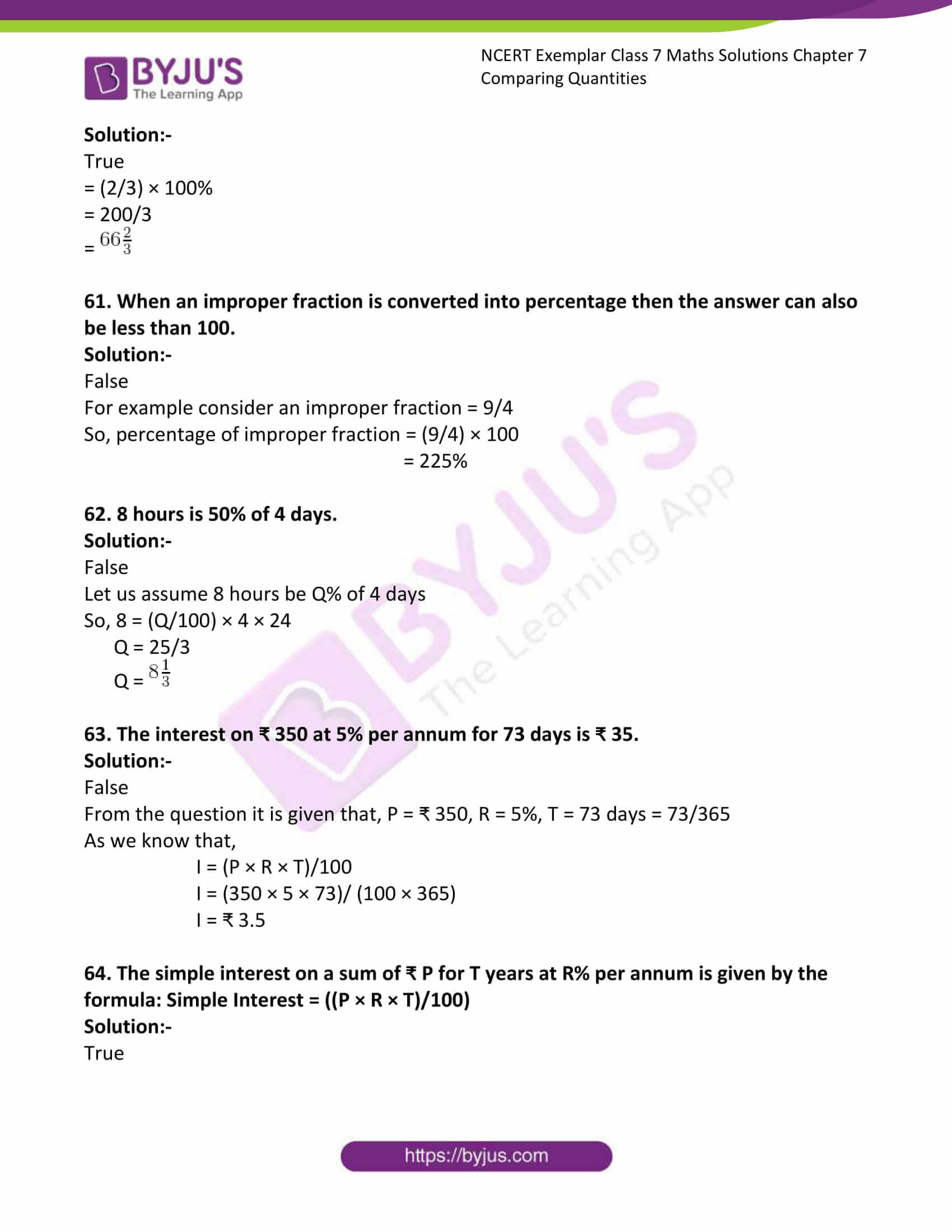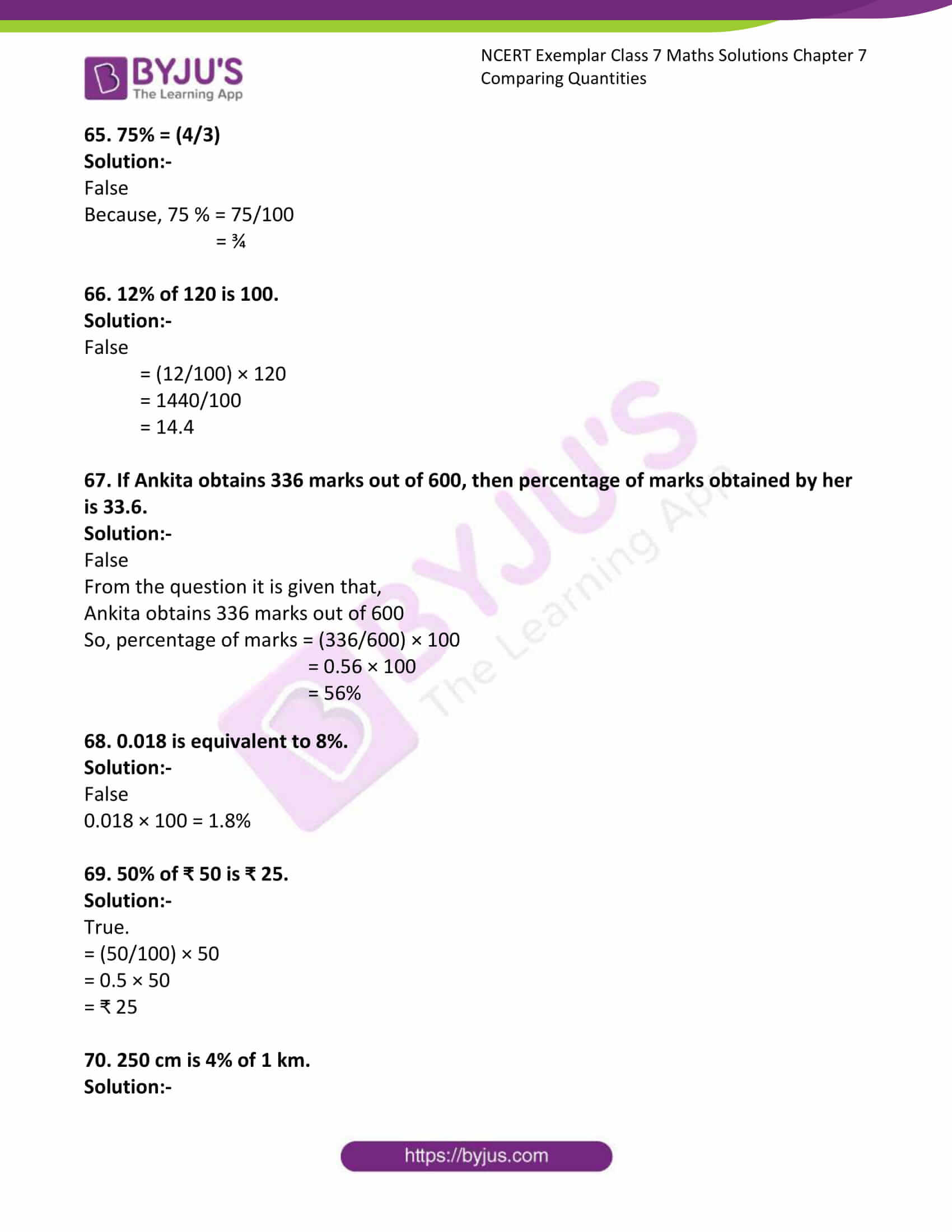### Access Answers to Maths NCERT Exemplar Solutions for Class 7 Chapter 7 Comparing Quantities

Exercise Page: 196

In questions 1 to 23, there are four options, out of which one is correct. Write the correct one.

1. 20% of 700 m is

(a) 560 m (b) 70 m (c) 210 m (d) 140 m

Solution: –

(d) 140m

20% of 700 m

The above condition can be written as = (20/100) × 700

= 14000/100

= 140 m

2. Gayatri’s income ₹ 1,60,000 per year. She pays 15% of this as house rent and 10% of the remainder on her child’s education. The money left with her is

(a) ₹136000 (b) ₹120000 (c) ₹122400 (d) ₹14000

Solution: –

(c) ₹122400

From the question it is given that,

Gayatri’s income ₹ 1,60,000 per year

She pays 15% of this as house rent = 15% of ₹ 1,60,000

= (15/100) × 1,60,000

= ₹ 24000

10% of the remainder on her child’s education = 10% of remaining amount of her income

Remaining amount of her income = ₹ 1,60,000 – ₹ 24000

= ₹ 136000

Total amount of her child’s education = 10% of ₹ 136000

= (10/100) × 136000

= 1360000/100

= ₹ 13600

Then, money left with Gayatri = ₹ 1,60,000 – (₹ 24000 + ₹ 13600)

= ₹ 1, 60, 000 – ₹ 37,600

= ₹ 1,22,400

3. The ratio of Fatima’s income to her savings is 4 : 1. The percentage of money saved by her is :

(a) 20% (b) 25% (c) 40% (d) 80%

Solution:-

(a) 20%

Let assume the ratio if Fatima’s income to her savings be 4y : 1y.

Then, the percentage of money saved by her is = (her savings/(income + savings)) × 100

= (1y/4y + y) × 100

= 100/5

= 20

4. 0.07 is equal to

(a) 70% (b) 7% (c) 0.7% (d) 0.07%

Solution:-

(b) 7%

0.07 = 7/100

Percentage = (7/100) × 100

= 7%

5. In a scout camp, 40% of the scouts were from Gujarat State and 20% of these were from Ahmedabad. The percentage of scouts in the camp from Ahmedabad is:

(a) 25 (b) 32.5 (c) 8 (d) 50

Solution:-

(c) 8

From the question it is given that,

In a scout camp, 40% of the scouts were from Gujarat State

So, let us assume number of scouts in the camp be 100

Then, scouts from Gujarat = 40% of 100

= (40/100) × 100

= 4000/100

= 40

Now, 20% of scouts were from Ahmedabad = 20% of 40

= (20 /100) × 40

= 800/100

= 8

Therefore, the percentage of scouts in the camp from Ahmedabad is 8.

6. What percent of ₹ 4500 is ₹ 9000?

(a) 200 (b) ½ (c) 2 (d) 50

Solution:-

(a) 200

Let us assume percentage be Q,

Then, Q% of ₹ 4500 is ₹ 9000

(Q/100) × 4500 = ₹ 9000

(4500Q/100) = ₹ 9000

45Q = ₹ 9000

Q = ₹ 9000/45

Q = ₹ 200

7. 5.2 is equal to

(a) 52% (b) 5.2% (c) 520% (d) 0.52%

Solution:-

(c) 520%

5.2 = (52/10) × 100

= (5200/10)

= 520%

8. The ratio 3 : 8 is equal to

(a) 3.75% (b) 37.5% (c) 0.375% (d) 267%

Solution:-

(b) 37.5%

The ratio 3 : 8 = (3/8) × 100

= 300/8

= 37.5%

9. 225% is equal to

(a) 9 : 4 (b) 4 : 9 (c) 3 : 2 (d) 2 : 3

Solution:-

(a) 9 : 4

225% = (225/100)

Because, to remove the % we have to divide the given number by 100.

= 9/4

10. A bicycle is purchased for ₹ 1800 and is sold at a profit of 12%. Its selling price is

(a) ₹ 1584 (b) ₹ 2016 (c) ₹ 1788 (d) ₹ 1812

Solution:-

(b) ₹ 2016

From the question it is given that,

A bicycle is purchased for ₹ 1800

Bicycle is sold at a profit of 12%.

Its selling price is =?

We know that, Profit percent = (Profit/CP) × 100

12 = (Profit/₹ 1800) × 100

(12 × 1800)/100 = Profit

Profit = 12 × 18

Profit = ₹ 216

Then, Profit = SP – CP

216 = SP – 1800

SP = 216 + 1800

SP = ₹ 2016

11. A cricket bat was purchased for ₹ 800 and was sold for ₹ 1600. Then profit earned is

(a) 100% (b) 64% (c) 50% (d) 60%

Solution:-

(a) 100%

From the question it is given that,

Cost price of bat (CP) = ₹ 800

Selling price of bat (SP) = ₹ 1600

Then, Profit = SP – CP

= 1600 – 800

= ₹ 800

We know that, Profit percent = (Profit/CP) × 100

= (800/800) × 100

= 100%

12. A farmer bought a buffalo for ₹ 44000 and a cow for ₹ 18000. He sold the buffalo at a loss of 5% but made a profit of 10% on the cow. The net result of the transaction is

(a) loss of ₹ 200 (b) profit of ₹ 400

(c) loss of ₹ 400 (d) profit of ₹ 200

Solution:-

(c) loss of ₹ 400

From the question it is given that,

Cost price of cow = ₹ 18000

Profit percent on cow = 10%

We know that, Profit percent = (Profit/CP) × 100

10 = (Profit/₹ 18000) × 100

(10 × 18000)/100 = Profit

Profit = 10 × 180

Profit = ₹ 1800

Then, Profit = SP – CP

1800 = SP – 18000

SP = 1800 + 18000

SP of cow = ₹ 19800

Cost price of buffalo = ₹ 44000

He sold the buffalo at a loss of 5%

We know that, Loss percent = (loss/CP) × 100

5 = (loss/44000) × 100

(5 × 44000)/100 = loss

Loss = 5 × 440

Loss = ₹ 2200

Loss = CP – SP

₹2200 = ₹44000 – SP

SP = 44000 – 2200

SP of buffalo = ₹ 41,800

Now,

Total cost price of both buffalo and cow = ₹ 44000 + ₹ 18000

= ₹ 62000

Total selling price of both buffalo and cow = ₹ 41800 + ₹ 19800

= ₹ 61,600

Selling price of both buffalo and cow is less when compare to cost price,

So, Loss = CP – SP

= ₹ 62000 – ₹ 61600

Loss = ₹ 400

13. If Mohan’s income is 25% more than Raman’s income, then Raman’s income is less than Mohan’s income by

(a) 25% (b) 80% (c) 20% (d) 75%

Solution:-

(c) 20%

Let us assume Mohan’s income be ‘P’ and Raman’s income be ‘Q’

From the question it is given that,

Mohan’s income is 25% more than Raman’s income i.e. x = y + 25% of y

X = y + (25/100) y

X = y (1 + (25/100))

X = y ((100 + 25)/100)

X = y(125/100)

Then, the difference between both the income is = (125/100)y – (25/100)y

So,

Raman’s income as compared to Mohan’s income,

= (difference)/ (Mohan’s income) × 100

= ((25y/100)/(125y/100)) × 100

= (25/125) × 100

= 20%

14. The interest on ₹ 30000 for 3 years at the rate of 15% per annum is

(a) ₹ 4500 (b) ₹ 9000 (c) ₹ 18000 (d) ₹ 13500

Solution:-

(d) ₹ 13500

From the question it is given that,

Principal= ₹ 30000

Time = 3 Years

Rate = 15%

Then, we know the formula of Simple interest I = (P × R × T)/100

I= (30000 × (15) × 3)/100

I = (30000 × 15 × 3)/100

I = ₹ 13500

15. Amount received on ₹ 3000 for 2 years at the rate of 11% per annum is

(a) ₹ 2340 (b) ₹ 3660 (c) ₹ 4320 (d) ₹ 3330

Solution:-

(b) ₹ 3660

From the question it is given that,

Principal= ₹ 3000

Time = 2 Years

Rate = 11%

Then, we know the formula of Simple interest I = (P × R × T)/100

I= (3000 × (11) × 2)/100

I = (3000 × 11 × 2)/100

I = ₹ 660

Amount = P + I

= 3000 + 660

= ₹ 3660

16. Interest on ₹ 12000 for 1 month at the rate of 10 % per annum is

(a) ₹ 1200 (b) ₹ 600 (c) ₹ 100 (d) ₹ 12100

Solution:-

(c) ₹ 100

From the question it is given that,

Principal= ₹ 12000

Time = 1 month = 1/12

Rate = 10%

Then, we know the formula of Simple interest I = (P × R × T)/100

I= (12000 × (10) × (1/12))/100

I = ₹ 100

17. Rajini and Mohini deposited ₹ 3000 and ₹ 4000 in a company at the rate of 10% per annum for 3 years and 2½ years respectively. The difference of the amounts received by them will be

(a) ₹ 100 (b) ₹ 1000

(c) ₹ 900 (d) ₹ 1100

Solution:-

(d) ₹ 1100

From the question it is given that,

Money deposited by Rajini = ₹ 3000

Time = 3 years

Rate = 10%

Then, we know the formula of Simple interest I = (P × R × T)/100

I= (3000× (10) × 3)/100

I = ₹ 900

Amount = P + I

= 3000 + 900

= ₹ 3900

Now,

Money deposited by Mohini = ₹ 4000

Time = 2½ = 5/2

Rate = 10%

Then, we know the formula of Simple interest I = (P × R × T)/100

I= (4000× (10) × (5/2))/100

I = ₹ 1000

Amount = P + I

= 4000 + 1000

= ₹ 5000

The difference of the amounts received by them will be = 5000 – 3900

= ₹1100

18. If 90% of x is 315 km, then the value of x is

(a) 325 km (b) 350 km (c) 405 km (d) 340 km

Solution:-

(b) 350 km

From the question it is given that,

90% of x is 315 km, it can be written as, (90/100) × x = 315

x = (315 × 100)/90

x = 31500/90

x = 350 km

19. On selling an article for ₹ 329, a dealer lost 6%. The cost price of the article is

(a) ₹ 310.37 (b) ₹ 348.74 (c) ₹ 335 (d) ₹ 350

Solution:-

(d) ₹ 350

From the question it is given that,

Selling price of the article = ₹ 329

Loss percent = 6%

We know that, Loss percent = (loss/CP) × 100

6 = ((CP – SP)/CP) × 100

(6/100)CP = CP –SP

CP – (6/100)CP = 329

(94/100)CP = 329

CP = (329 × 100)/94

CP = ₹ 350

20. (25% of 50% of 100%)/(25 × 50) is equal to

(a) 1.1% (b) 0.1% (c) 0.01% (d) 1%

Solution:-

(c) 0.01%

= [(25/100) × (50/100) × (100/100)]/ (25 × 50)

= [¼ × ½ × 1]/(1250)

= [1/8] × (1/1250)

= 1/10000

= 0.0001 × 100

= 0.01%

21. The sum which will earn a simple interest of ₹ 126 in 2 years at 14% per annum is

(a) ₹ 394 (b) ₹ 395 (c) ₹ 450 (d) ₹ 540

Solution:-

(c) ₹ 450

From the question it is given that,

SI = ₹ 126

Time = 2 years

Rate = 14%

Then, we know the formula of Simple interest I = (P × R × T)/100

126 = (P × 14 × 2)/100

P = 12600/(14 × 2)

P = ₹ 450

22. The per cent that represents the unshaded region in the figure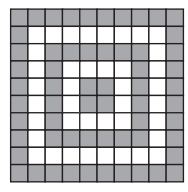(a) 75% (b) 50% (c) 40% (d) 60%

Solution:-

(c) 40%

From the figure,

Total number of parts in the given figure = 10 × 10 = 100

Out of 100 parts 60 parts are shaded.

So, unshaded parts = 100 – 60 = 40 parts

Then percentage of unshaded parts = (40/100) × 100

= 0.4 × 100

= 40%

23. The per cent that represents the shaded region in the figure is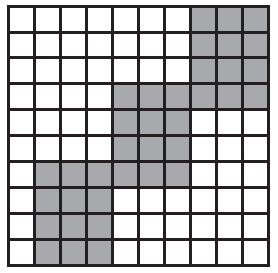(a) 36% (b) 64% (c) 27% (d) 48%

Solution:-

(a) 36%

From the figure,

Total number of parts in the given figure = 10 × 10 = 100

Out of 100 parts 36 parts are shaded.

Then percentage of shaded parts = (36/100) × 100

= 0.36 × 100

= 36%

In each of the questions 24 to 59, fill in the blanks to make the statements true.

24. 2 : 3 = ________ %

Solution:-

2: 3 =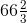%

= (2/3) × 100

=%

25. 18¾% = :

Solution:-

18¾% = 3 : 16

18¾% = 75/4 %

= (75/4) × (1/100)

= 3: 16

26. 30% of ₹ 360 = ________.

Solution:-

30% of ₹ 360 = ₹ 108.

It can be written as = (30/100) × 360

= 10800/100

= ₹ 108

27. 120 % of 50 km = ________.

Solution:-

120 % of 50 km = 60 km.

It can be written as = (120/100) × 50

= 6000/100

= 60 km

28. 2.5 = ________%

Solution:-

2.5 = 250 %

2.5 = 2.5 × 100

= 250%

29. (8/5) = %

Solution:-

(8/5) = 160 %.

(8/5) is written in percentage as = (8/5) × 100

= 8 × 20

= 160 %

30. A _______ with its denominator 100 is called a percent.

Solution:-

A fraction with its denominator 100 is called a percent.

31. 15 kg is _______ % of 50 kg.

Solution:-

15 kg is 30 % of 50 kg.

Let us assume the percentage be P.

So, P% of 50 kg =15 kg

(P/100) × 50 = 15

P = (15 × 100)/50

P = 1500/50

P = 150/5

P = 30

32. Weight of Nikhil increased from 60 kg to 66 kg. Then, the increase in weight is _______ %.

Solution:-

Weight of Nikhil increased from 60 kg to 66 kg. Then, the increase in weight is 10 %.

From the question it is given that, Weight of Nikhil increased from 60 kg to 66 kg.

So, weight increase = 66 – 60

= 6 kg

Then, percentage increase = (Weight increase/Initial weight) × 100%

= (6/60) × 100

= 600/60

= 60/6

= 10%

33. In a class of 50 students, 8 % were absent on one day. The number of students present on that day was ________.

Solution:-

In a class of 50 students, 8 % were absent on one day. The number of students present on that day was 46.

From the question it is given that, number of students in the class = 50

Percentage of students were absent on one day = 8%

Then, percentage of students were present on one day = 100% – 8%

= 92%

So, 92% of 50

= (92/100) × 50

= 4600/100

= 46 students

34. Savitri obtained 440 marks out of 500 in an examination. She secured _______ % marks in the examination.

Solution:-

Savitri obtained 440 marks out of 500 in an examination. She secured 88% marks in the examination.

From the question it is give that, Savitri obtained 440 marks out of 500 in an examination,

Then, percentage of marks obtained by Savitri = (440/500) × 100

= 0.88 × 100

= 88%

35. Out of a total deposit of ₹ 1500 in her bank account, Abida withdrew 40% of the deposit. Now the balance in her account is ______.

Solution:-

Out of a total deposit of ₹ 1500 in her bank account, Abida withdrew 40% of the deposit. Now the balance in her account is ₹ 900.

From the question,

Total deposit in Abida’s account = ₹ 1500

Percentage of amount Abida withdrew = 40%

Then, amount withdrew = 40% of ₹ 1500

= (40/100) × 1500

= 60000/100

= ₹ 600

Then, remaining amount in the account = ₹ 1500 – ₹ 600

= ₹ 900

36. ________ is 50% more than 60.

Solution:-

90 is 50% more than 60.

Let us assume the number be Q.

From the question, Q is 50% more than 60

So, Q = 60 + (50/100) × 60

= 60 + (3000/100)

= 60 + 30

= 90

37. John sells a bat for ₹ 75 and suffers a loss of ₹ 8. The cost price of the bat is________.

Solution:-

John sells a bat for ₹ 75 and suffers a loss of ₹ 8. The cost price of the bat is ₹ 83.

From the question it is given that, Selling price of bat ₹ 75

Loss = ₹ 8

We know that, Cost Price (CP) = SP + loss

= ₹ 75 + ₹ 8

= ₹ 83

38. If the price of sugar is decreased by 20%, then the new price of 3kg sugar originally costing ₹ 120 will be

Solution:-

If the price of sugar is decreased by 20%, then the new price of 3kg sugar originally costing ₹ 120 will be ₹ 96

From the question it is given that, price of 3kg sugar originally costing ₹ 120

The price of the sugar is decreased by 20%

Then, the new price of sugar = 120 – 20% of original price

= 120 – (20/100) × 120

= 120 – (2400/100)

= 120 – 24

= ₹ 96

39. Mohini bought a cow for ₹ 9000 and sold it at a loss of ₹ 900. The selling price of the cow is ________.

Solution:-

Mohini bought a cow for ₹ 9000 and sold it at a loss of ₹ 900. The selling price of the cow is ₹ 8100

From the question it is given that,

Cost price of cow (CP) = ₹ 9000

Loss = ₹ 900

We know that, Selling price (SP) = CP – loss

= 9000 – 900

= ₹ 8100

40. Devangi buys a chair for ₹ 700 and sells it for ₹ 750. She earns a profit of ________ % in the transaction.

Solution:-

Devangi buys a chair for ₹ 700 and sells it for ₹ 750. She earns a profit of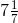% in the transaction.

From the question it is given that, cost price of chair = ₹ 700

As we know that, Profit = SP – CP

= 750 – 700

= ₹ 50

Then, Profit percentage = (profit/CP) × 100%

= (50/700) × 100

=%

41. Sonal bought a bed sheet for ₹ 400 and sold it for ₹ 440. Her ________% is ________.

Solution:-

Sonal bought a bed sheet for ₹ 400 and sold it for ₹ 440. Her Profit% is 10.

From the question, it is given that, cost price of bed = ₹ 400

Selling price of bed = ₹ 440

SP is more than CP so, Profit = SP – CP

= 440 – 400

= ₹ 40

We know that, Profit percentage = (Profit/CP) × 100

= (40/400) × 100

= 10%

42. Nasim bought a pen for ₹ 60 and sold it for ₹ 54. His ________% is ________.

Solution:-

Nasim bought a pen for ₹ 60 and sold it for ₹ 54. His loss% is 10.

From the question, it is given that, cost price of pen = ₹ 60

Selling price of pen = ₹ 54

CP is more than SP so, Loss = CP – SP

= 60 – 54

= ₹ 6

We know that, loss percentage = (loss/CP) × 100

= (6/60) × 100

= 10%

43. Aahuti purchased a house for ₹ 50,59,700 and spent ₹ 40300 on its repairs. To make a profit of 5%, she should sell the house for ₹ ________.

Solution:-

Aahuti purchased a house for ₹ 50,59,700 and spent ₹ 40300 on its repairs. To make a profit of 5%, she should sell the house for ₹ 5355000.

From the question it is give that,

Cost price of house purchased by Aahuti = ₹ 50,59,700

Amount spent to repair the house = ₹ 40,300

Then, total cost price of house = ₹ 50,59,700 + 40,300

= ₹ 5100000

Profit % = (profit/CP) × 100

5 = ((SP – CP)/CP) × 100

5 = ((SP – 5100000)/5100000) × 100

(5 × 5100000)/100 = SP – 5100000

SP = ₹ 5355000

44. If 20 lemons are bought for ₹ 10 and sold at 5 for three rupees, then ________ in the transaction is ________%.

Solution:-

If 20 lemons are bought for ₹ 10 and sold at 5 for three rupees, then profit in the transaction is 20%.

From the question, it is given that, cost price of 20 lemons = ₹ 10

Selling price of 5 lemons = ₹ 3

So, selling price of 1 lemon = ₹ 3/5

Then, selling price of 20 lemons = ₹ (3/5) × 20

= 12

SP is more than CP so, Profit = SP – CP

= 12 – 10

= ₹ 2

We know that, Profit percentage = (Profit/CP) × 100

= (2/10) × 100

= 20%

45. Narain bought 120 oranges at ₹ 4 each. He sold 60 % of the oranges at ₹ 5 each and the remaining at ₹ 3.50 each. His ________ is ________%.

Solution:-

Narain bought 120 oranges at ₹ 4 each. He sold 60 % of the oranges at ₹ 5 each and the remaining at ₹ 3.50 each. His profit is 10%.

From the question, it is given that, cost price of one orange = ₹ 4

cost price of 120 oranges = ₹ 4 × 120 = ₹ 480

So, selling price of 60% of oranges = ₹ 5 per each

60% of 120 oranges = (60/100) × 120

= 7200/100

= 72 oranges

Selling price of 72 oranges = 72 × 5 = ₹ 360

SP of remaining oranges = (120 – 72) × 3.50

= 48 × 3.50

= ₹ 168

Therefore total selling price of 120 oranges = ₹ 360 + ₹ 168

= ₹ 528

SP is more than CP so, Profit = SP – CP

= 528 – 480

= ₹ 48

We know that, Profit percentage = (Profit/CP) × 100

= (48/480) × 100

= 10%

46. A fruit seller purchased 20 kg of apples at ₹ 50 per kg. Out of these, 5% of the apples were found to be rotten. If he sells the remaining apples at ₹ 60 per kg, then his _________is _________%.

Solution:-

A fruit seller purchased 20 kg of apples at ₹ 50 per kg. Out of these, 5% of the apples were found to be rotten. If he sells the remaining apples at ₹ 60 per kg, then his profit is 14 %.

From the question it is given that, A fruit seller purchased 20 kg of apples at ₹ 50 per kg

In that 20 kg of apples 5% were rotten, so good apples = 20 – 5% of 20kg

= 20 – (5/100) × 20

= 20 – (100/100)

= 20 – 1

= 19 kg

So, 19 kg apples were sold at ₹ 60 per kg

Then, total Selling price of apples = 19 × 60

= ₹ 1140

Given cost price of 20 kg of apples = 20 × 50

= ₹ 1000

SP is more than CP so, Profit = SP – CP

= 1140 – 1000

= ₹ 140

We know that, Profit percentage = (Profit/CP) × 100

= (140/1000) × 100

= 14%

47. Interest on ₹ 3000 at 10% per annum for a period of 3 years is ________.

Solution:-

Interest on ₹ 3000 at 10% per annum for a period of 3 years is ₹ 900.

From the question it is given that,

Principal= ₹ 3000

Time = 3 years

Rate = 10%

Then, we know the formula of Simple interest I = (P × R × T)/100

I= (3000 × (10) × 3)/100

I = ₹ 900

48. Amount obtained by depositing ₹ 20,000 at 8 % per annum for six months is ________.

Solution:-

Amount obtained by depositing ₹ 20,000 at 8 % per annum for six months is ₹ 20800

From the question it is given that,

Principal= ₹ 20000

Time = 6 months = 6/12 = ½

Rate = 8%

Then, we know the formula of Simple interest I = (P × R × T)/100

I= (20000 × 8 × ½ ) /100

I = ₹ 800

Amount received = Principal + Interest

= 20000 + 800

= ₹ 20800

49. Interest on ₹ 12500 at 18% per annum for a period of 2 years and 4 months is ________.

Solution:-

Interest on ₹ 12500 at 18% per annum for a period of 2 years and 4 months is ₹ 5250.

From the question it is given that,

Principal= ₹ 12500

Time = 2 years 4 months = (2 + (4/12))

= (2 + (1/3))

= 7/3 year

Rate = 18%

Then, we know the formula of Simple interest I = (P × R × T)/100

I= (12500 × 18 × (7/3)) /100

I = ₹ 5250

50. 25 ml is _________ percent of 5 litres.

Solution:-

25 ml is 0.5 percent of 5 litres

Let us assume 25 ml be Q % of 5 liters.

So, 25 ml = Q % of 5l

25 = (Q/100) × 5 × 1000

Q = (25 × 100)/(5 × 1000)

Q = 0.5%

51. If A is increased by 20%, it equals B. If B is decreased by 50%, it equals C. Then __________ % of A is equal to C.

Solution:-

If A is increased by 20%, it equals B. If B is decreased by 50%, it equals C. Then 60 % of A is equal to C.

From the question it is given that, A is increased by 20%, it equals B = A + (20/100) × A

B is decreased by 50%, it equals C = B – (50/100) × B

Then,

A (1 + (1/5)) = B

(6/5) A = B … [equation i]

Now,

B (1 – (1/2)) = C

½B = C

B = 2C … [equation ii]

Substitute equation (ii) in equation (i)

(6/5)A = 2C

C = (6/10) A

C = ((6 × 10)/(10 × 10))A

C = (60/100) × A

C = 60% of A

52. Interest = (P × R × T)/100, where

T is

R % is and

P is .

Solution:-

Interest = (P × R × T)/100, where

T is Time period

R % is Rate of Interest and

P is Principal.

53. The difference of interest for 2 years and 3 years on a sum of ₹ 2100 at 8% per annum is _________.

Solution:-

The difference of interest for 2 years and 3 years on a sum of ₹ 2100 at 8% per annum is ₹ 168.

From the question it is given that,

P = ₹ 2100

Time = 2 years

Rate = 8%

Then, we know the formula of Simple interest I = (P × R × T)/100

I = (2100 × 2 × 8)/100

I = 33600/100

I = ₹ 336

Then,

Time = 3 years

I = (P × R × T)/100

I = (2100 × 3× 8)/100

I = 50400/100

I = ₹ 504

The difference of interest for 2 years and 3 years = 3 years – 2 years

= ₹ 504 – ₹ 336

= ₹ 168

54. To convert a fraction into a percent, we _________ it by 100.

Solution:-

To convert a fraction into a percent, we multiply it by 100.

55. To convert a decimal into a percent, we shift the decimal point two places to the _________.

Solution:-

To convert a decimal into a percent, we shift the decimal point two places to the right.

56. The _________ of interest on a sum of ₹ 2000 at the rate of 6% per annum for 1½ years and 2 years is ₹ 420.

Solution:-

The sum of interest on a sum of ₹ 2000 at the rate of 6% per annum for 1½ years and 2 years is ₹ 420.

From the question it is given that, P = 2000, R = 6% and T = 1½ years

As we know that,

I = (P × R × T)/100

I = (2000 × 6 × 3)/ (100 × 2)

I = ₹ 180

For 2 years,

I = (P × R × T)/100

I = (2000 × 6 × 2)/ (100)

I = ₹ 240

Then, sum = 180 + 240 = ₹ 420

57. When converted into percentage, the value of 6.5 is _________ than 100%.

Solution:-

When converted into percentage, the value of 6.5 is more than 100%.

Given, 6.5

= 6.5 × 100

= 650%

In questions 58 and 59, copy each number line. Fill in the blanks so that each mark on the number line is labelled with a percent, a fraction and a decimal. Write all fractions in lowest terms.

58.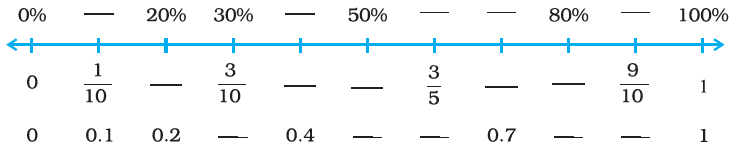Solution:-

We know that, Percentage = Fraction × 100

Then, Fraction = Percentage /100

Decimal = Percentage/ 100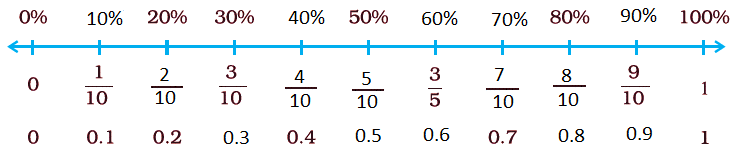59.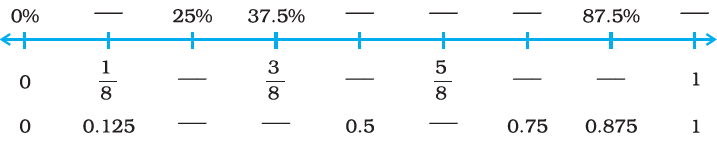Solution:-

We know that, Percentage = Fraction × 100

Then, Fraction = Percentage /100

Decimal = Percentage/ 100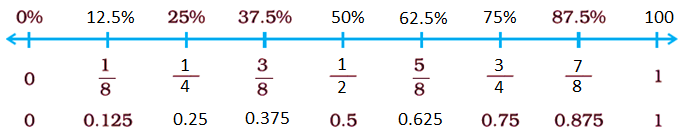In questions 60 to 79, state whether the statements are True or False.

60. (2/3) =Solution:-

True

= (2/3) × 100%

= 200/3

=61. When an improper fraction is converted into percentage then the answer can also be less than 100.

Solution:-

False

For example consider an improper fraction = 9/4

So, percentage of improper fraction = (9/4) × 100

= 225%

62. 8 hours is 50% of 4 days.

Solution:-

False

Let us assume 8 hours be Q% of 4 days

So, 8 = (Q/100) × 4 × 24

Q = 25/3

Q =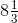63. The interest on ₹ 350 at 5% per annum for 73 days is ₹ 35.

Solution:-

False

From the question it is given that, P = ₹ 350, R = 5%, T = 73 days = 73/365

As we know that,

I = (P × R × T)/100

I = (350 × 5 × 73)/ (100 × 365)

I = ₹ 3.5

64. The simple interest on a sum of ₹ P for T years at R% per annum is given by the formula: Simple Interest = ((P × R × T)/100)

Solution:-

True

65. 75% = (4/3)

Solution:-

False

Because, 75 % = 75/100

= ¾

66. 12% of 120 is 100.

Solution:-

False

= (12/100) × 120

= 1440/100

= 14.4

67. If Ankita obtains 336 marks out of 600, then percentage of marks obtained by her is 33.6.

Solution:-

False

From the question it is given that,

Ankita obtains 336 marks out of 600

So, percentage of marks = (336/600) × 100

= 0.56 × 100

= 56%

68. 0.018 is equivalent to 8%.

Solution:-

False

0.018 × 100 = 1.8%

69. 50% of ₹ 50 is ₹ 25.

Solution:-

True.

= (50/100) × 50

= 0.5 × 50

= ₹ 25

70. 250 cm is 4% of 1 km.

Solution:-

False

250 cm = 250/100

= 2.5 m

We know that, 1 km = 1000m

Then, 4 % of 1 km = (4/100) × 1000

= 40 m